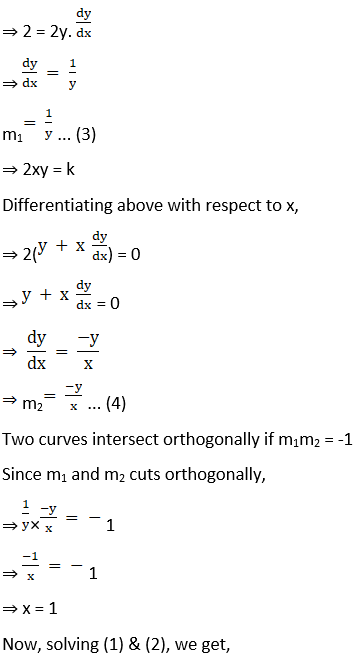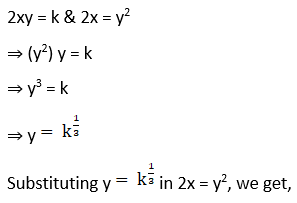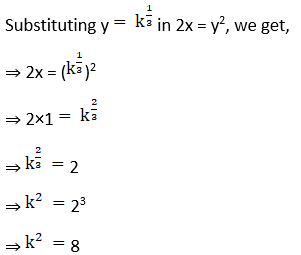# RD Sharma Solutions For Class 12 Maths Exercise 16.3 Chapter 16 Tangents and Normals

RD Sharma Solutions for Class 12 Maths Exercise 16.3 Chapter 16 Tangents and Normals are provided here. RD Sharma Solutions for Class 12 are available on the BYJU’S website in PDF format with solutions prepared by experienced faculty. This exercise explains the angle of intersection of two curves. If we draw tangents to these curves at the intersecting point, the angle between these tangents is called the angle between two curves.

The PDF of RD Sharma Solutions for Class 12 Maths Chapter 16 Tangents and Normals Exercise 16.3 are provided here. Some of the important topics of this exercise are listed below.

• Finding the equations of tangent and normal
• Finding the equation of the curve
• The angle of intersection of two curves
• Orthogonal curves

## RD Sharma Solutions For Class 12 Tangents and Normals Exercise 16.3: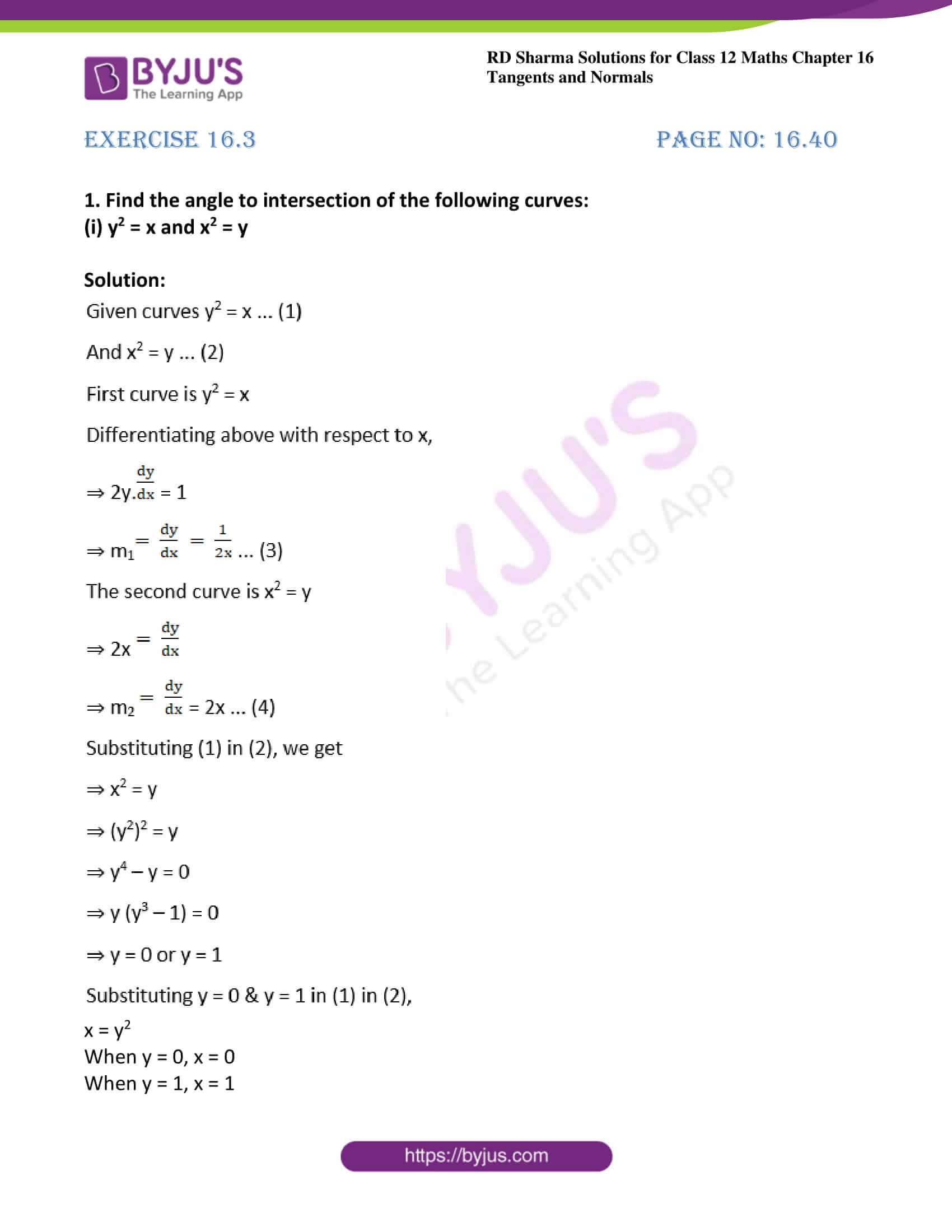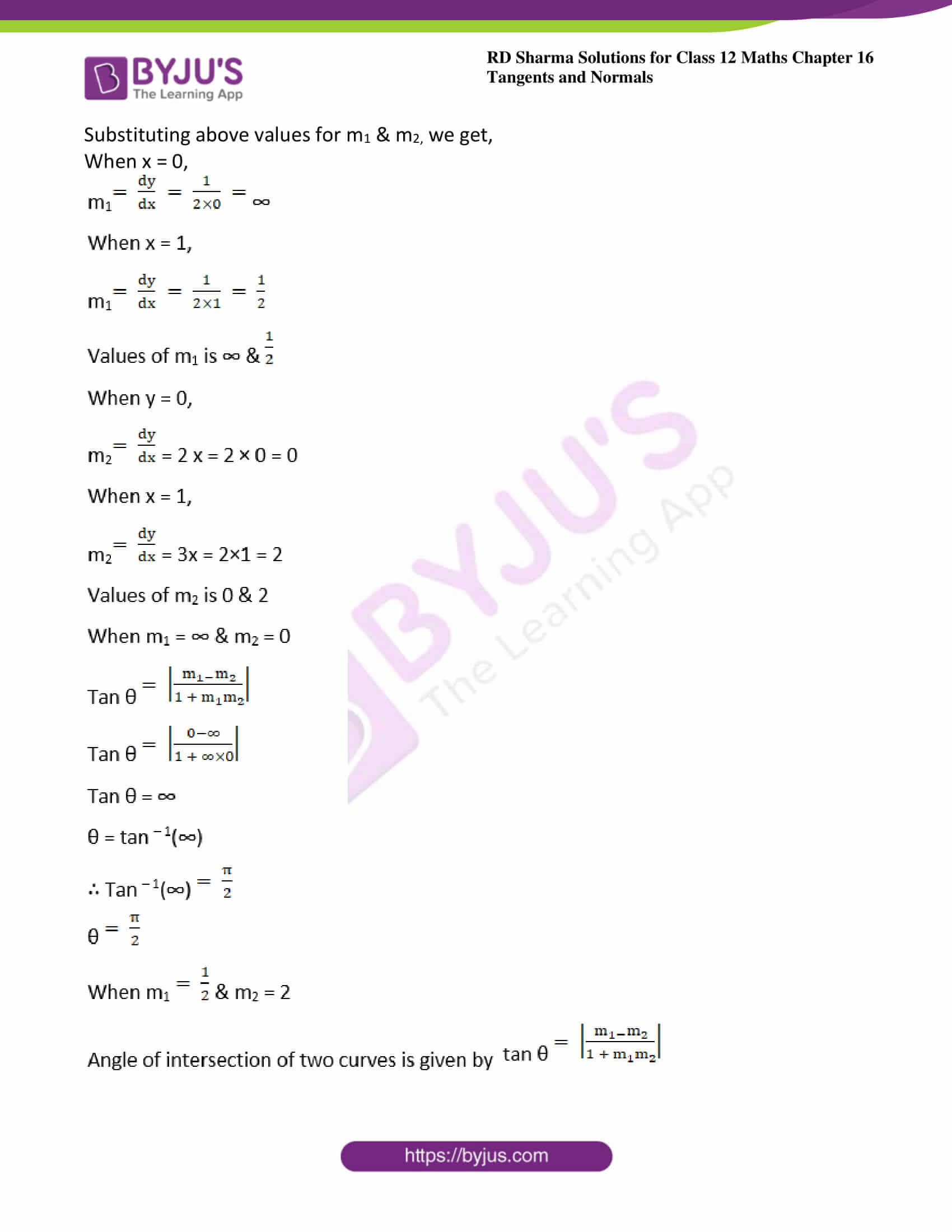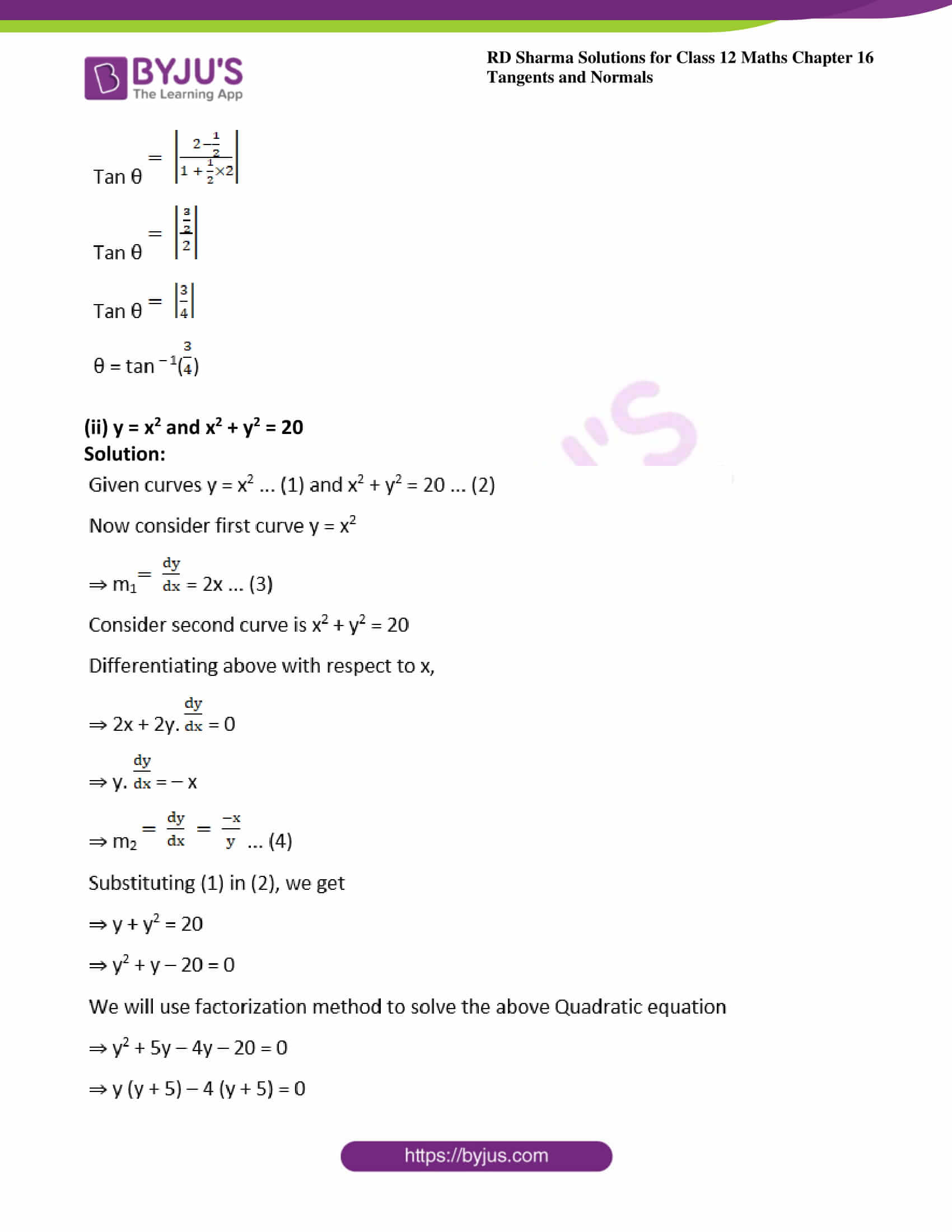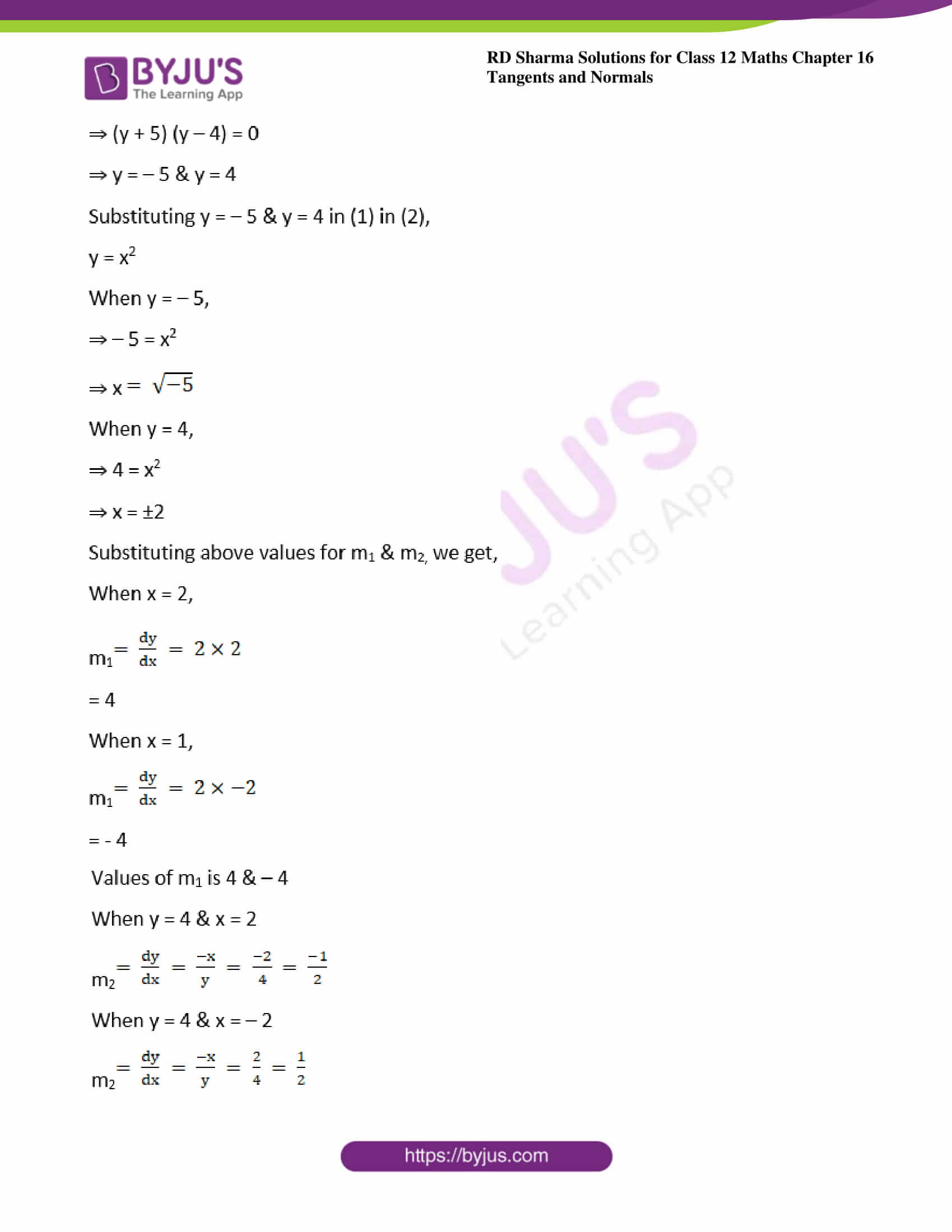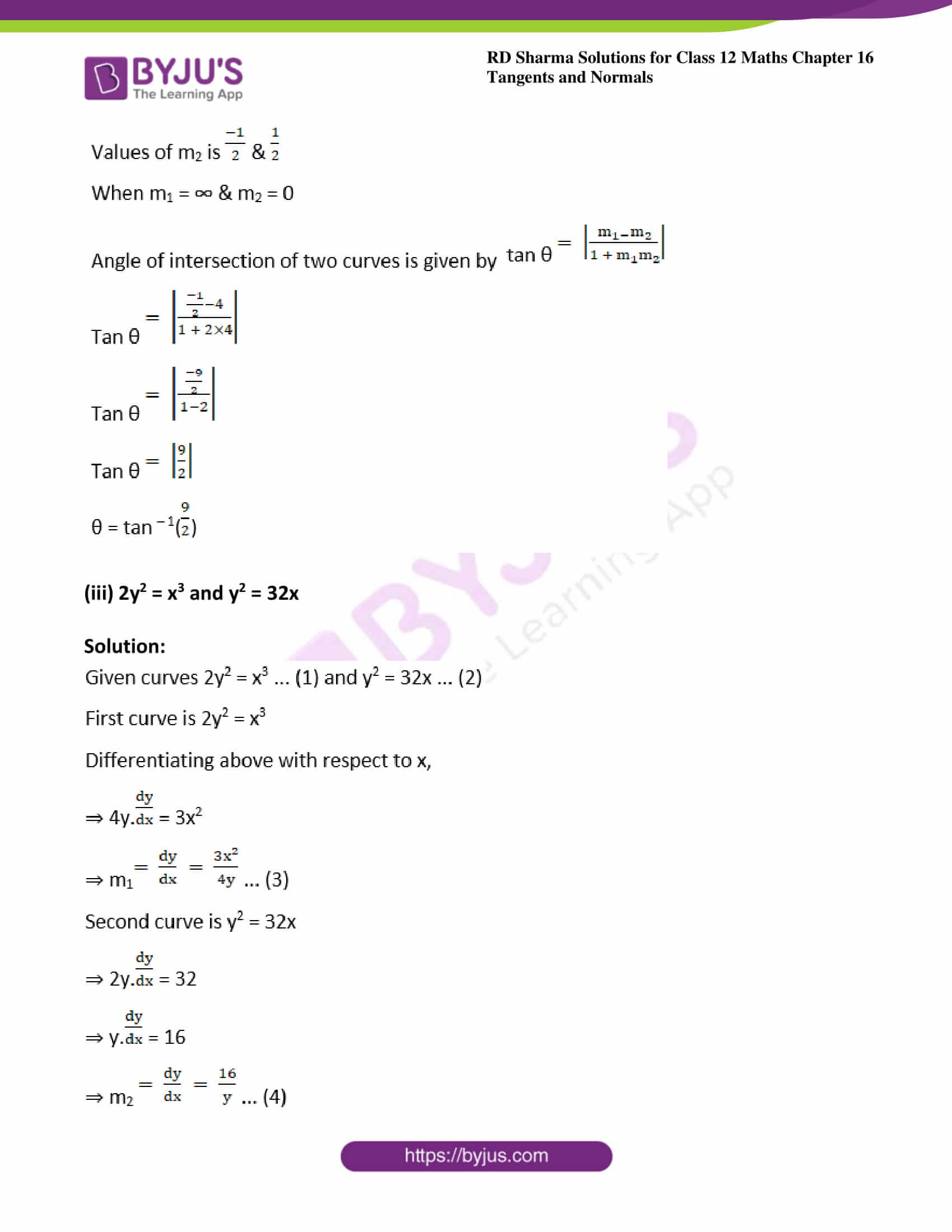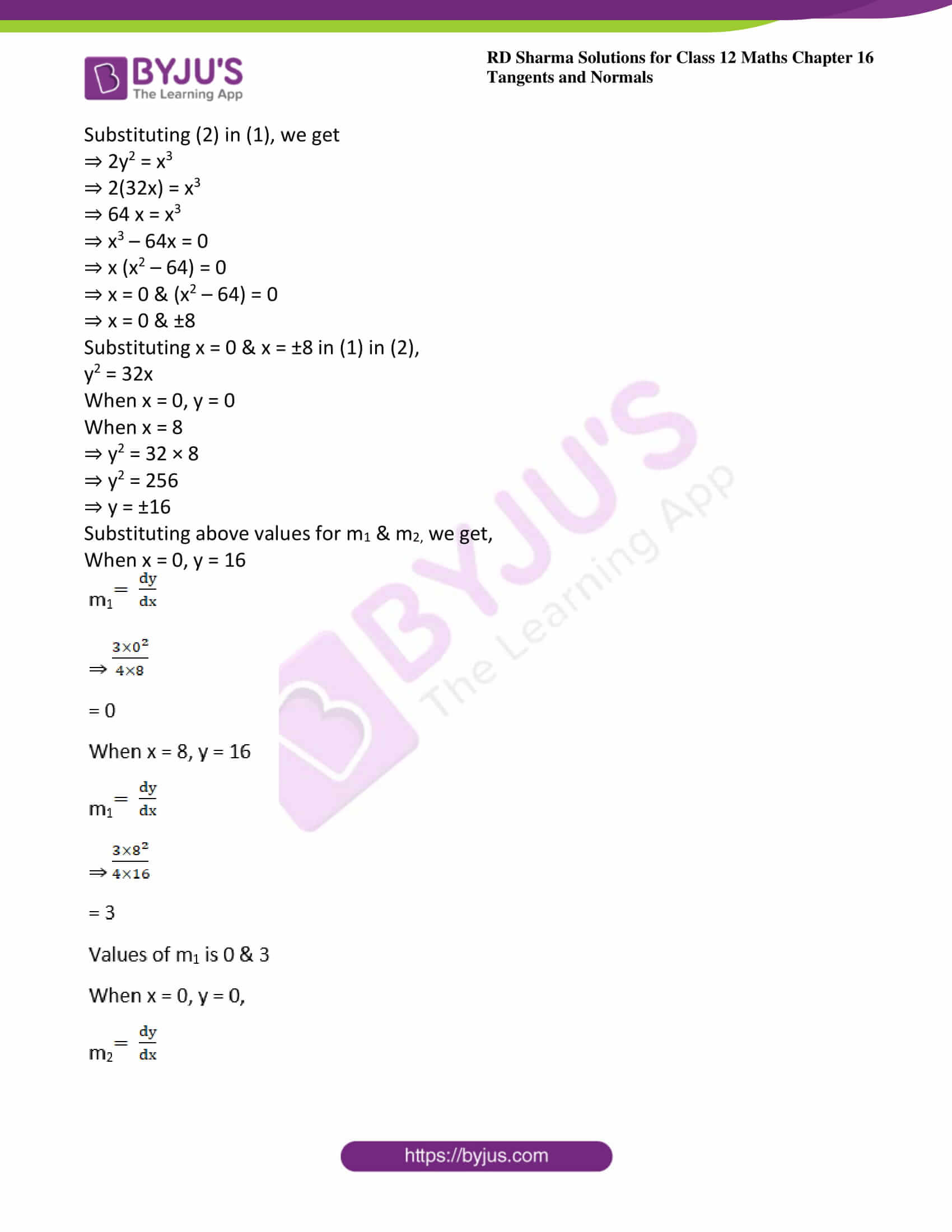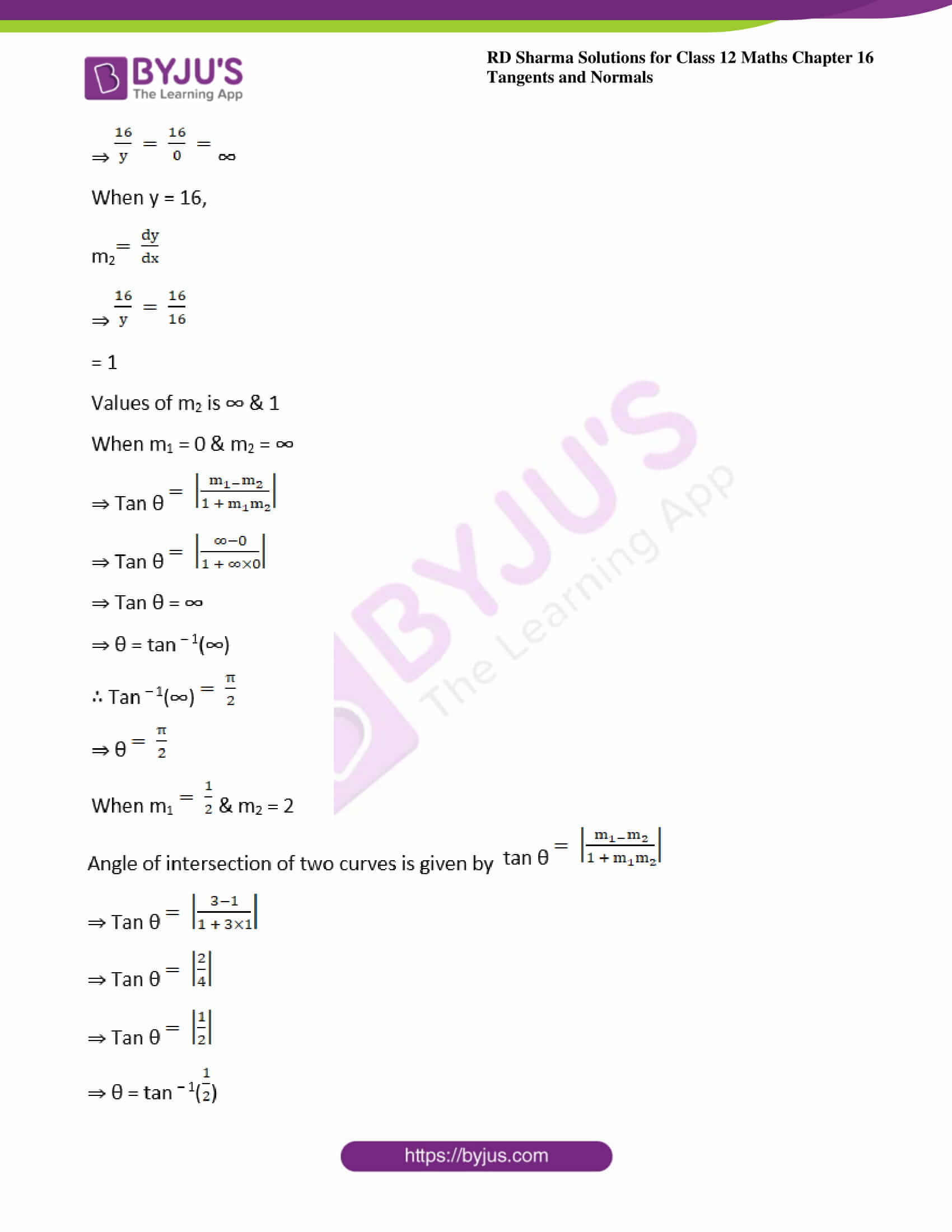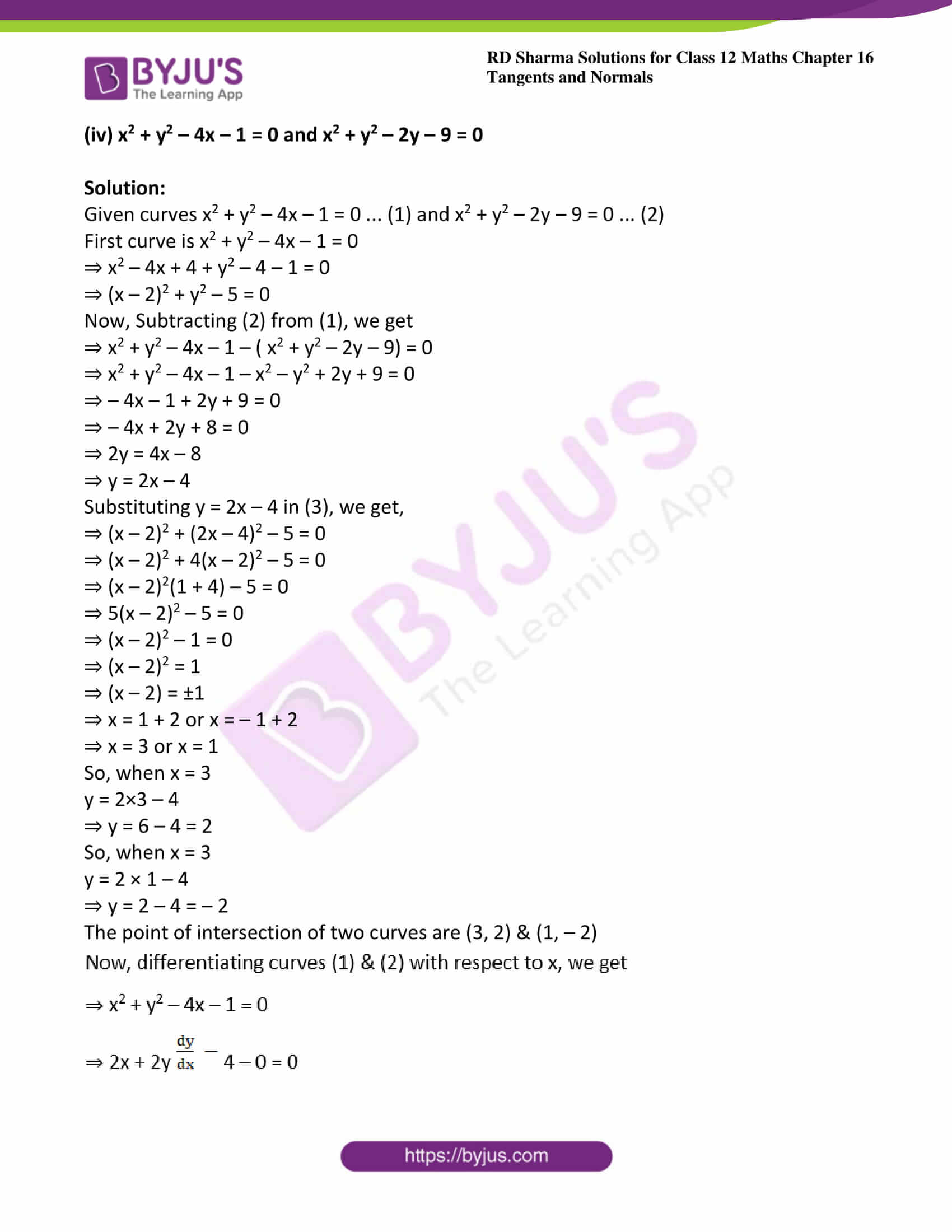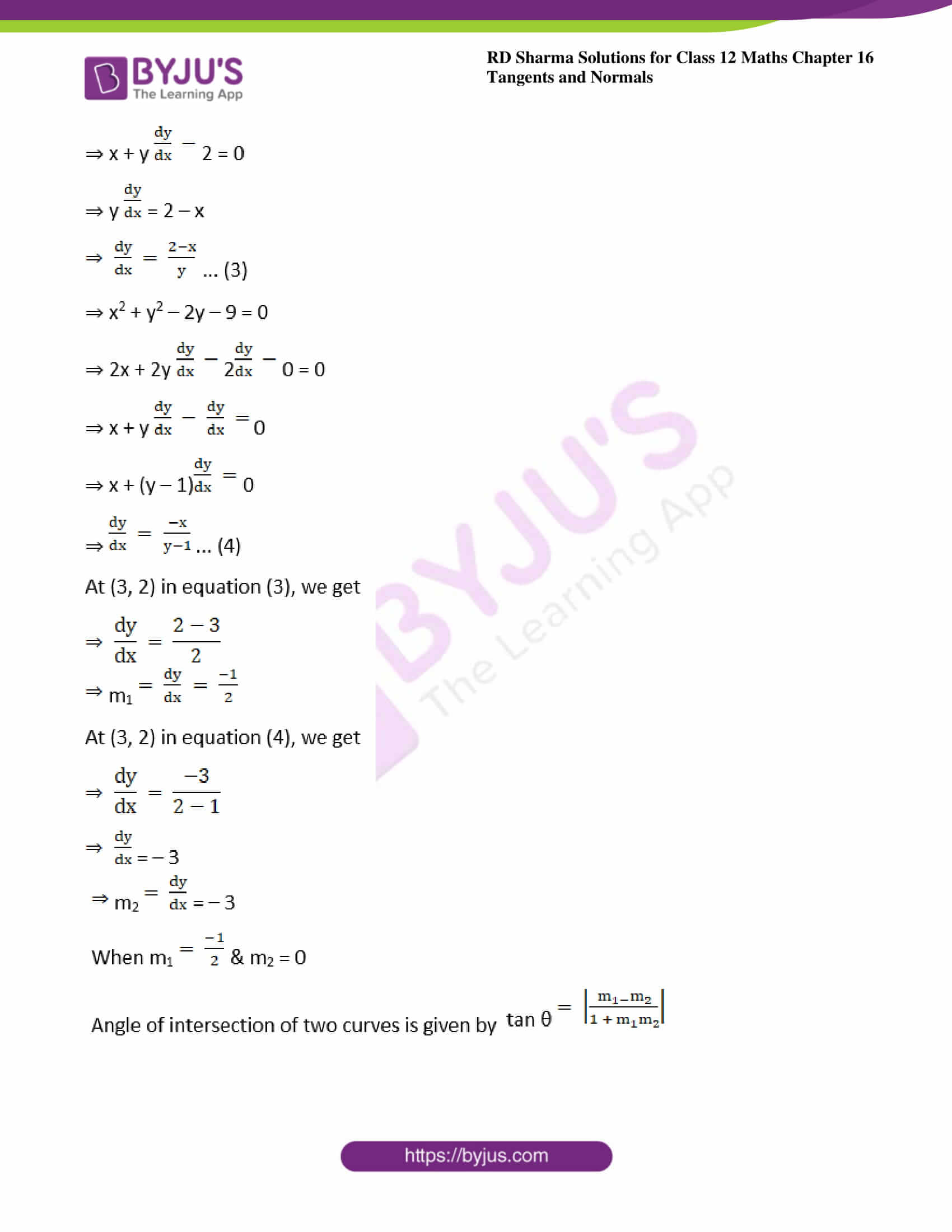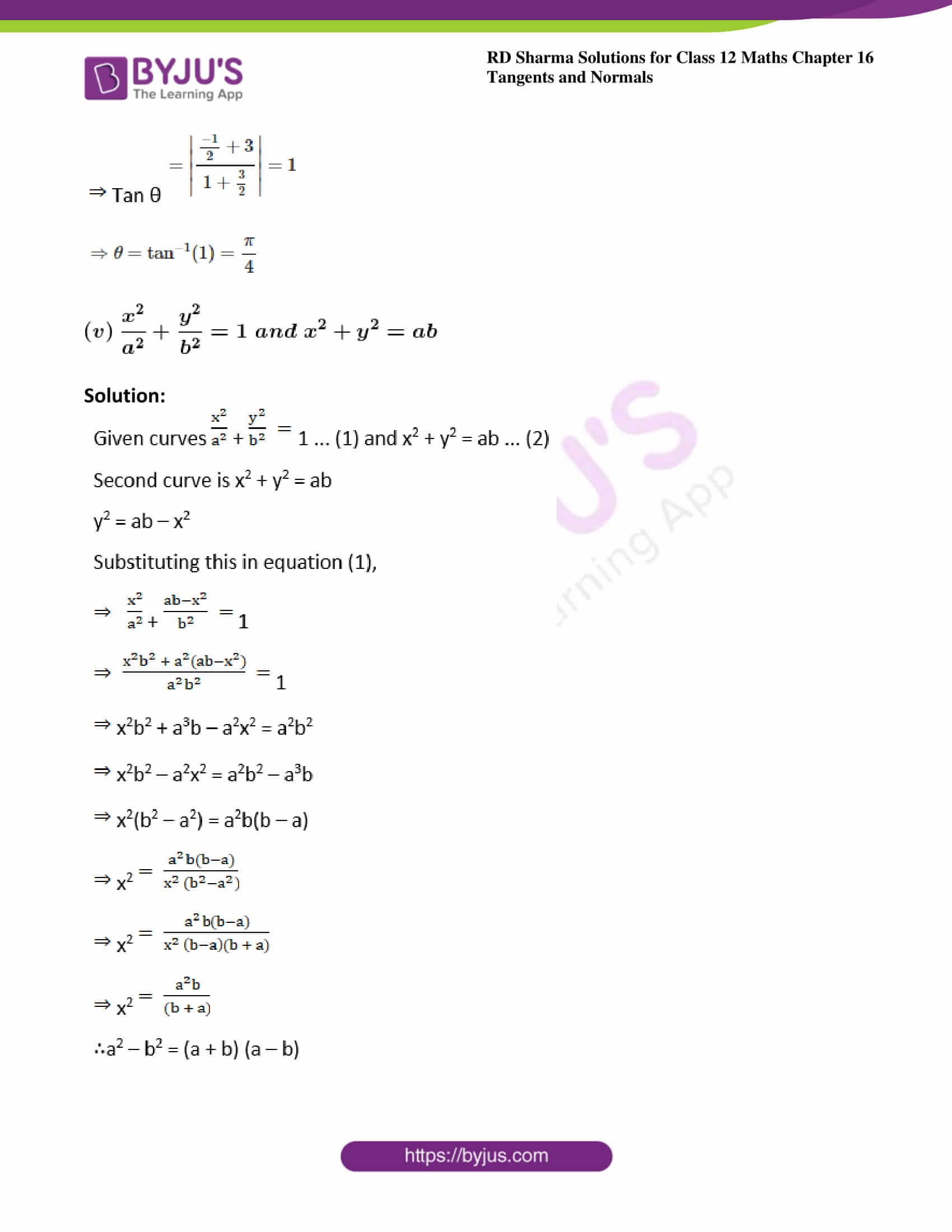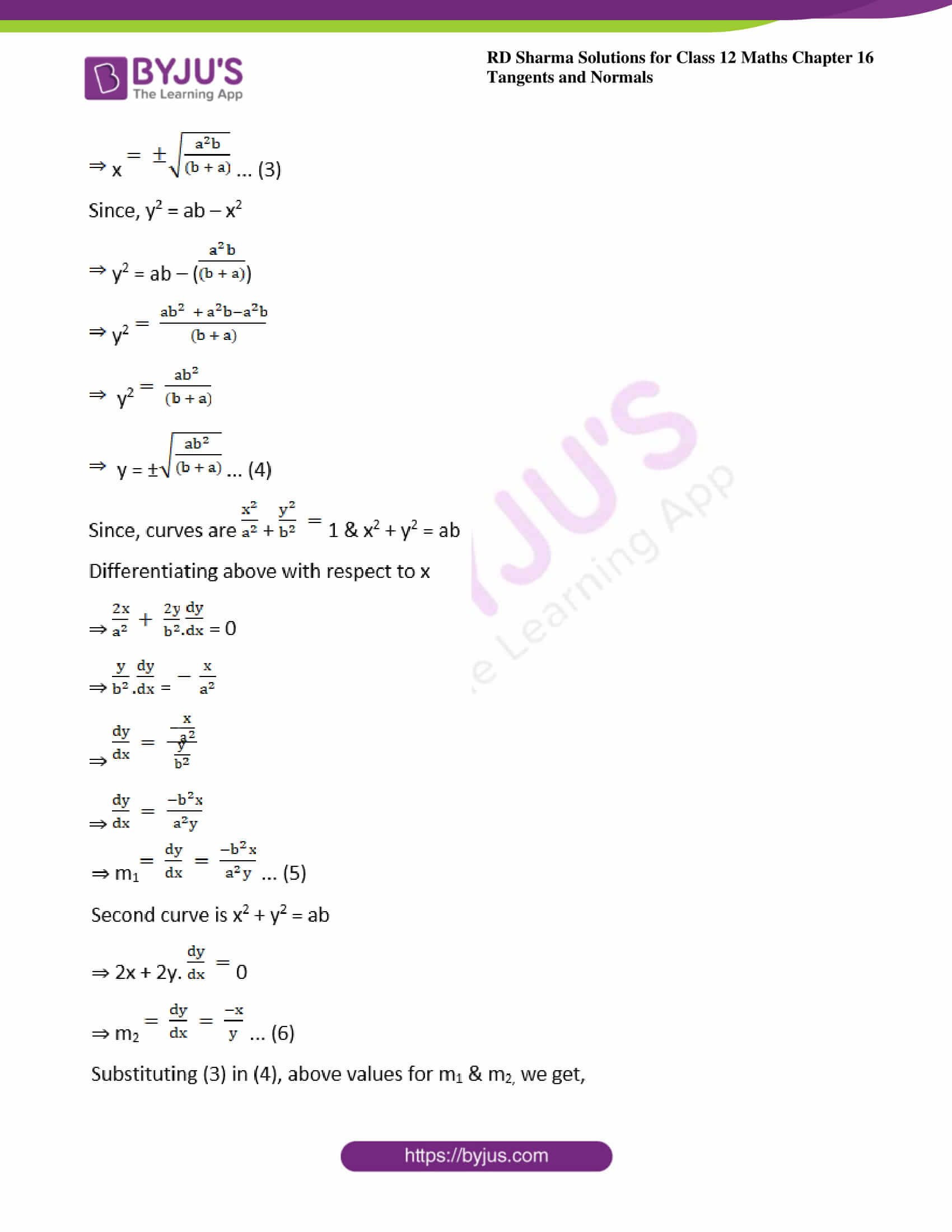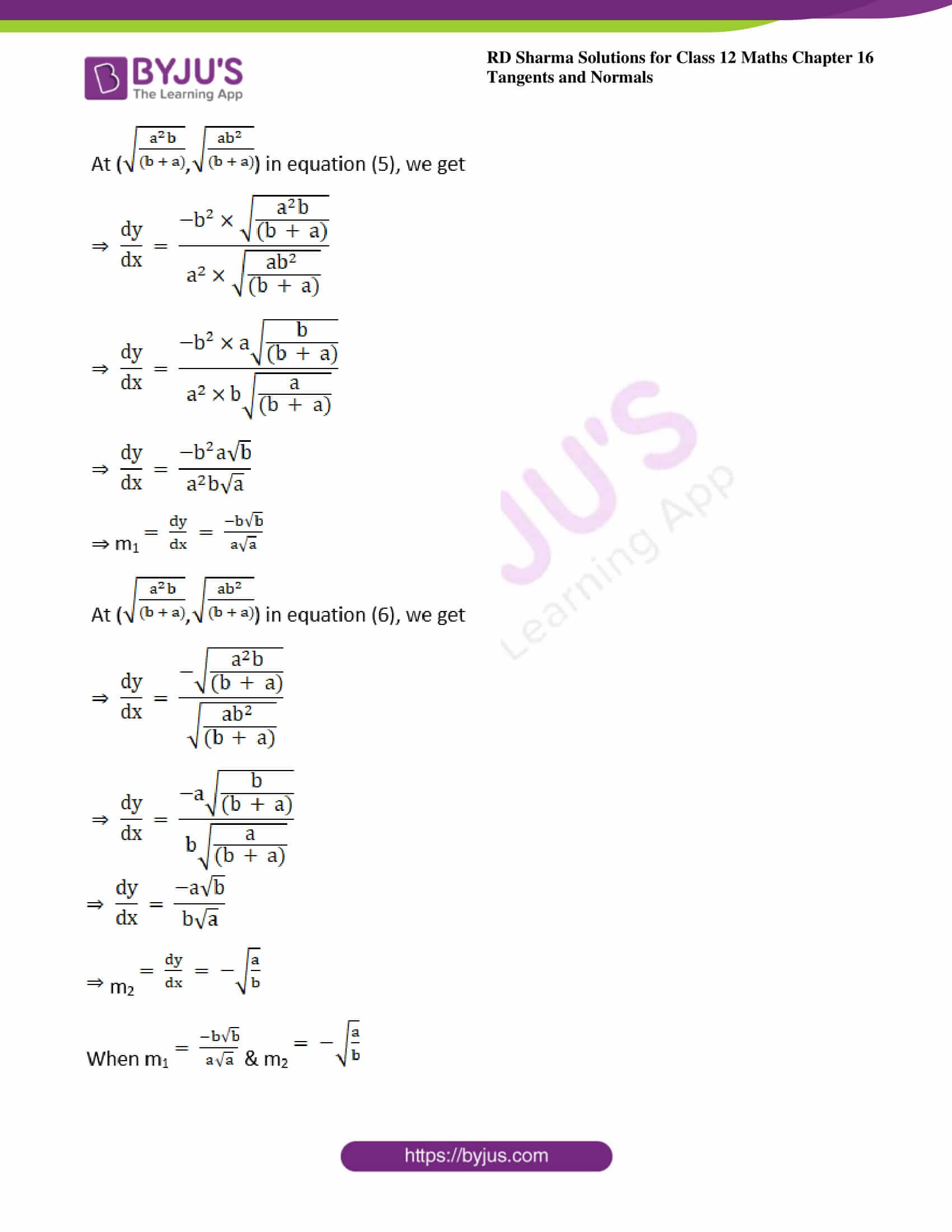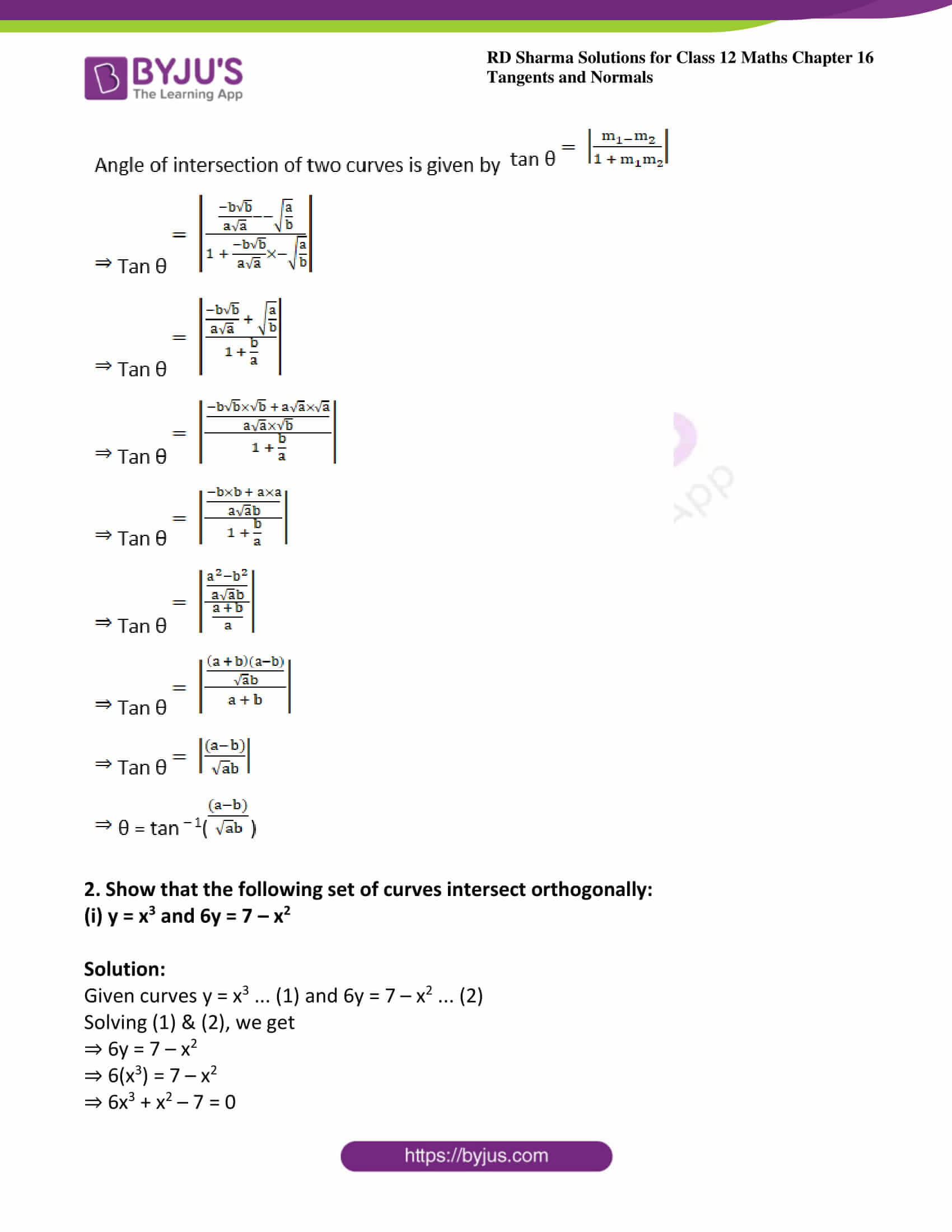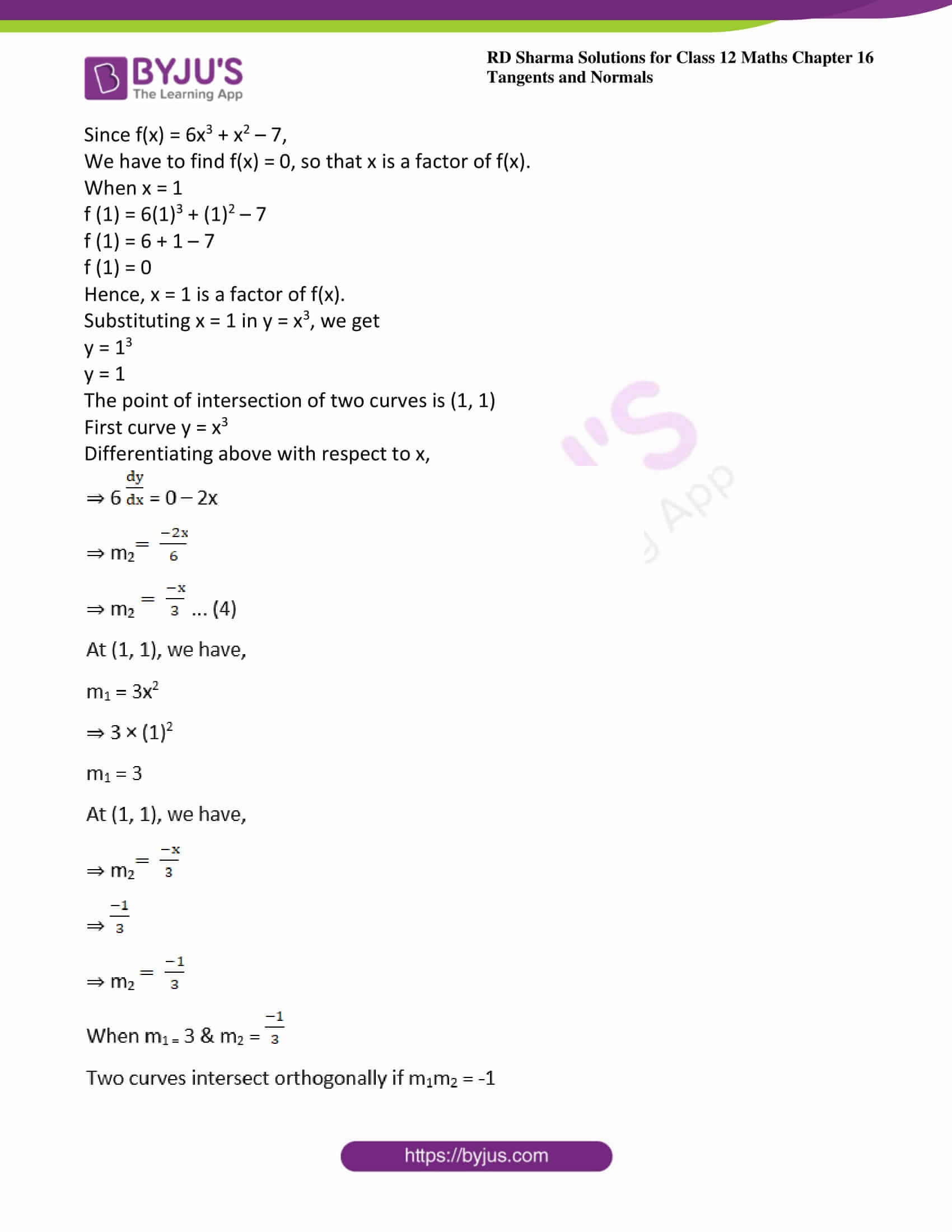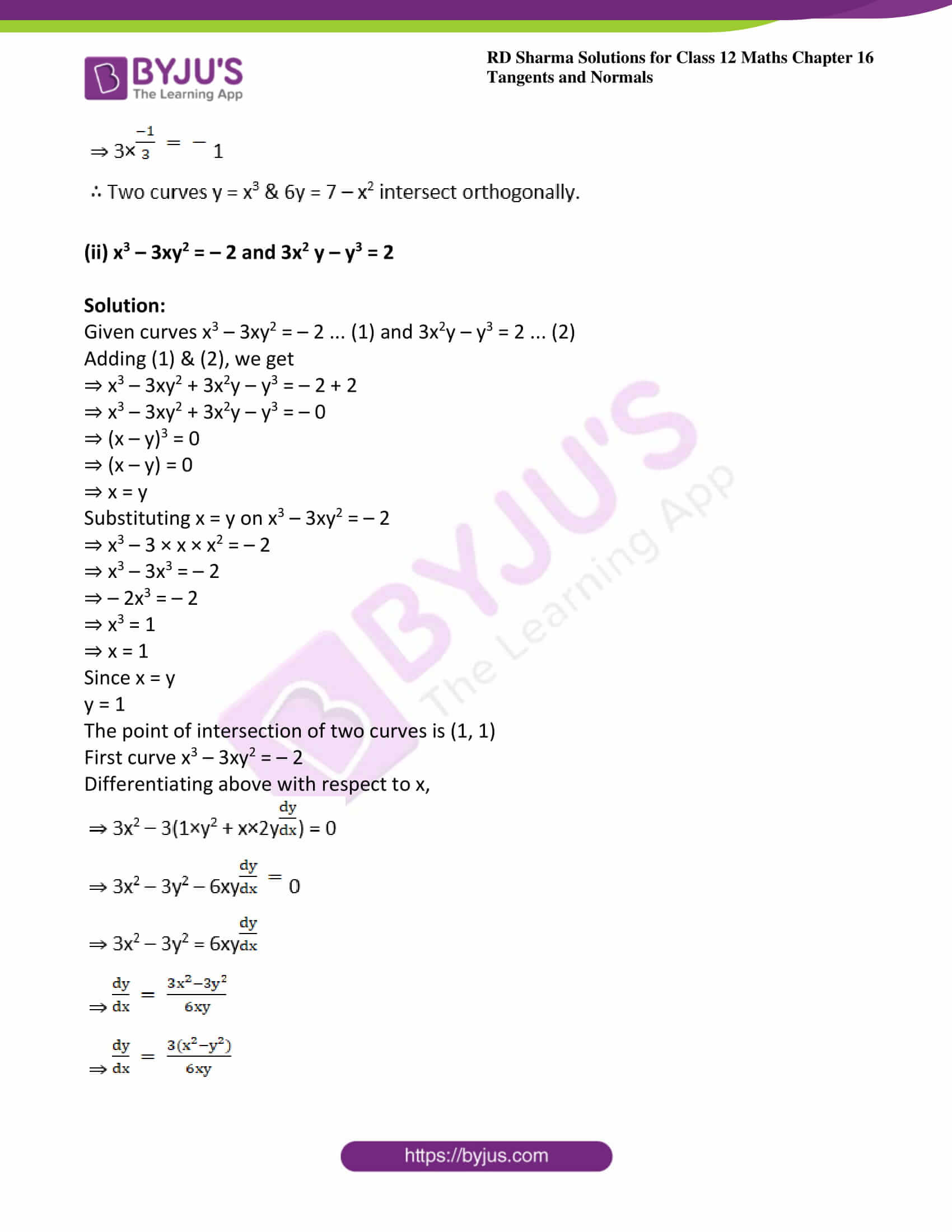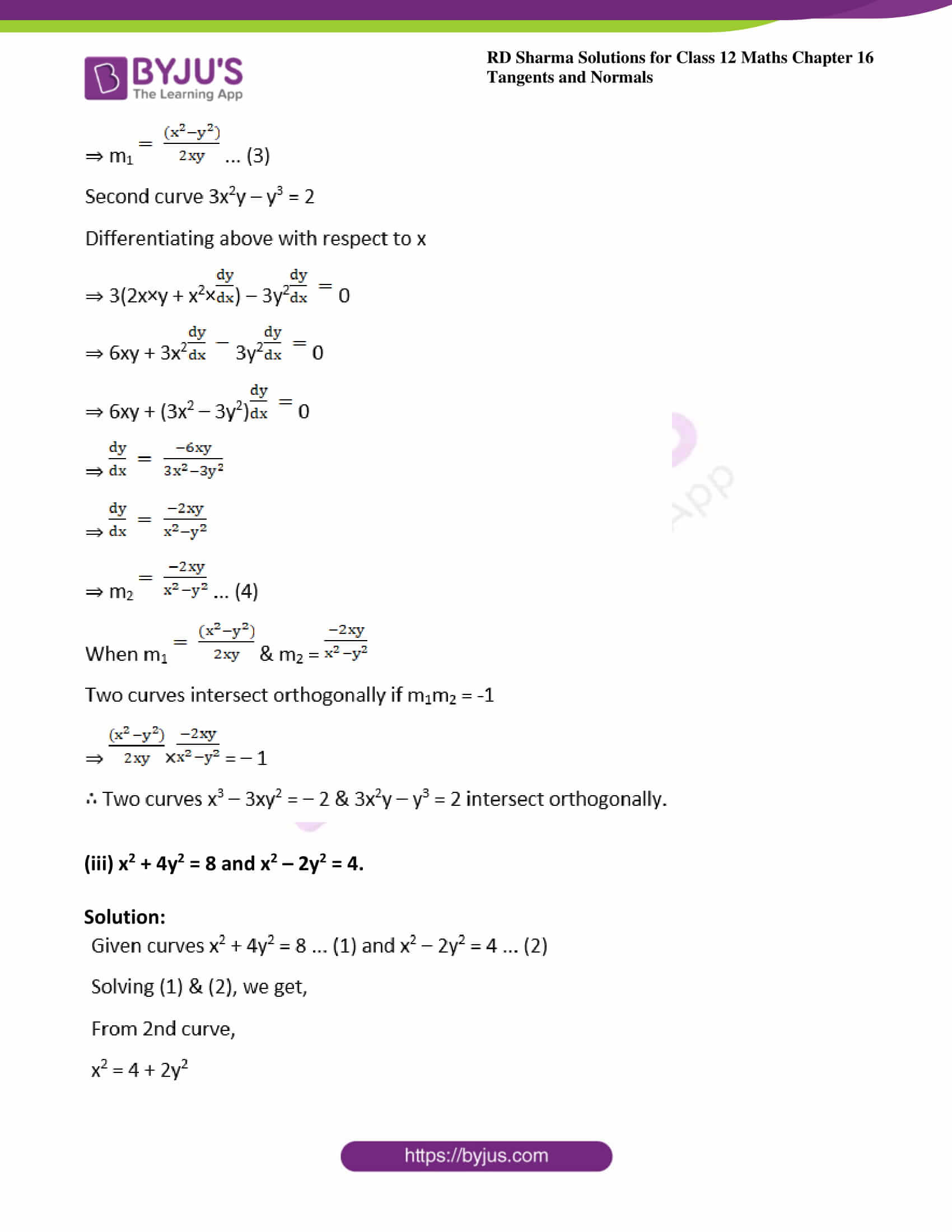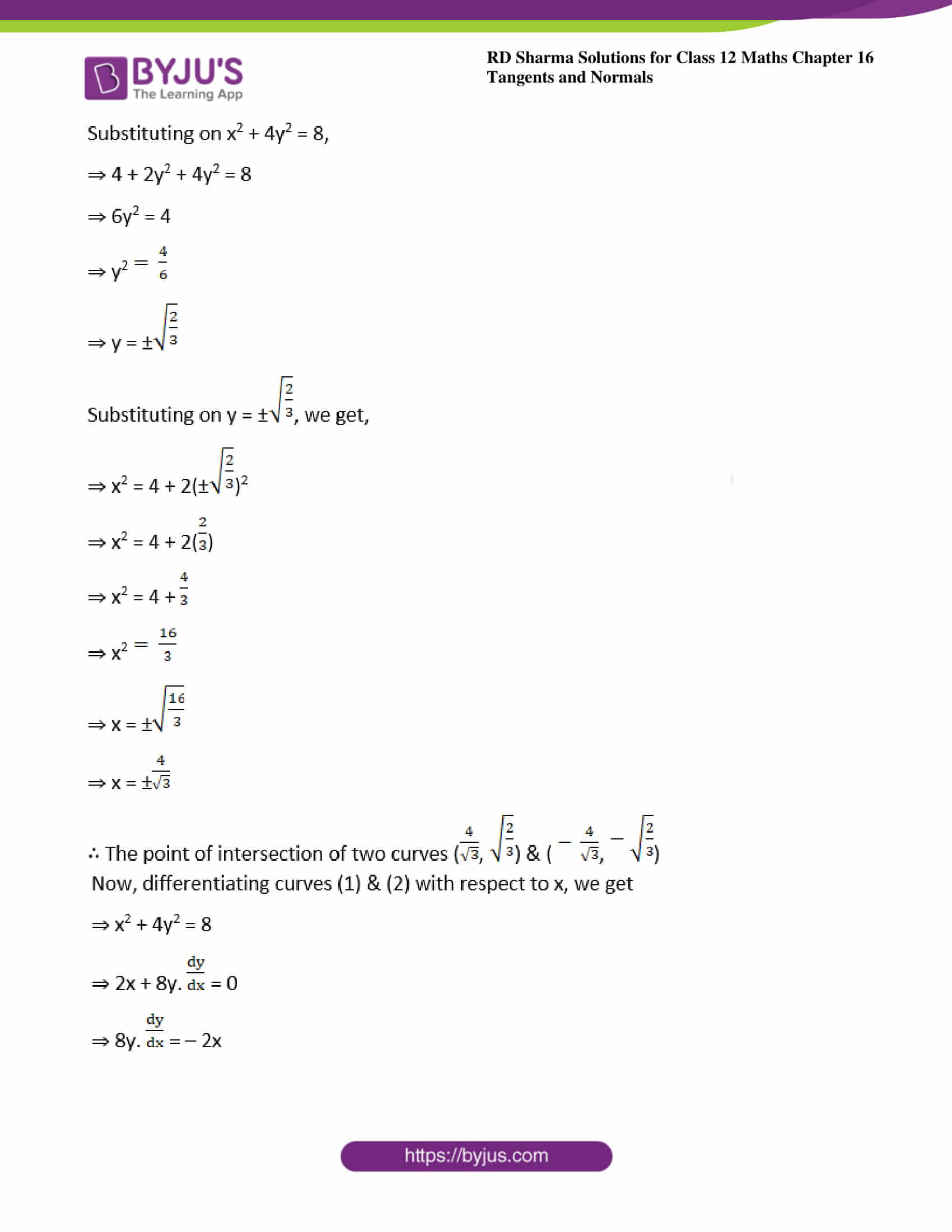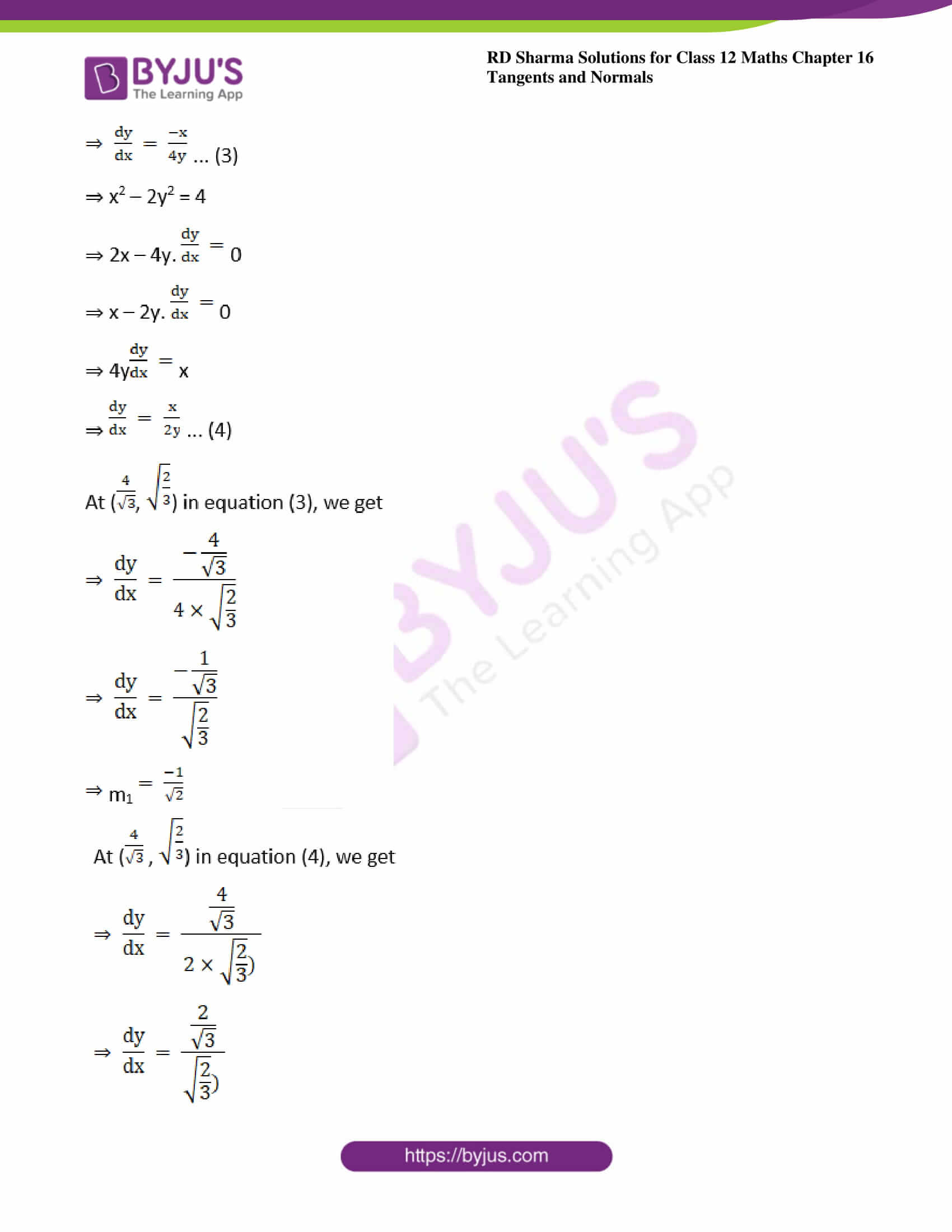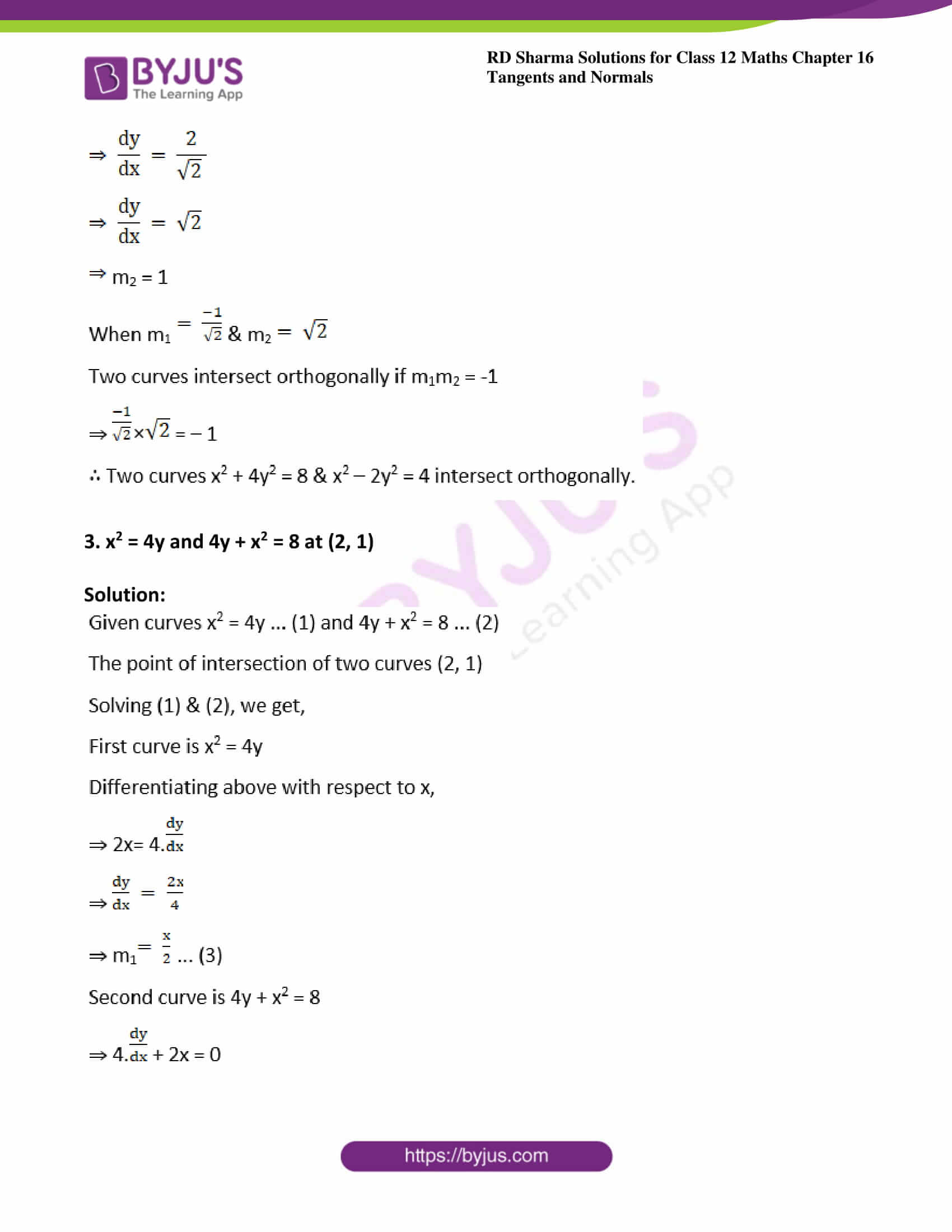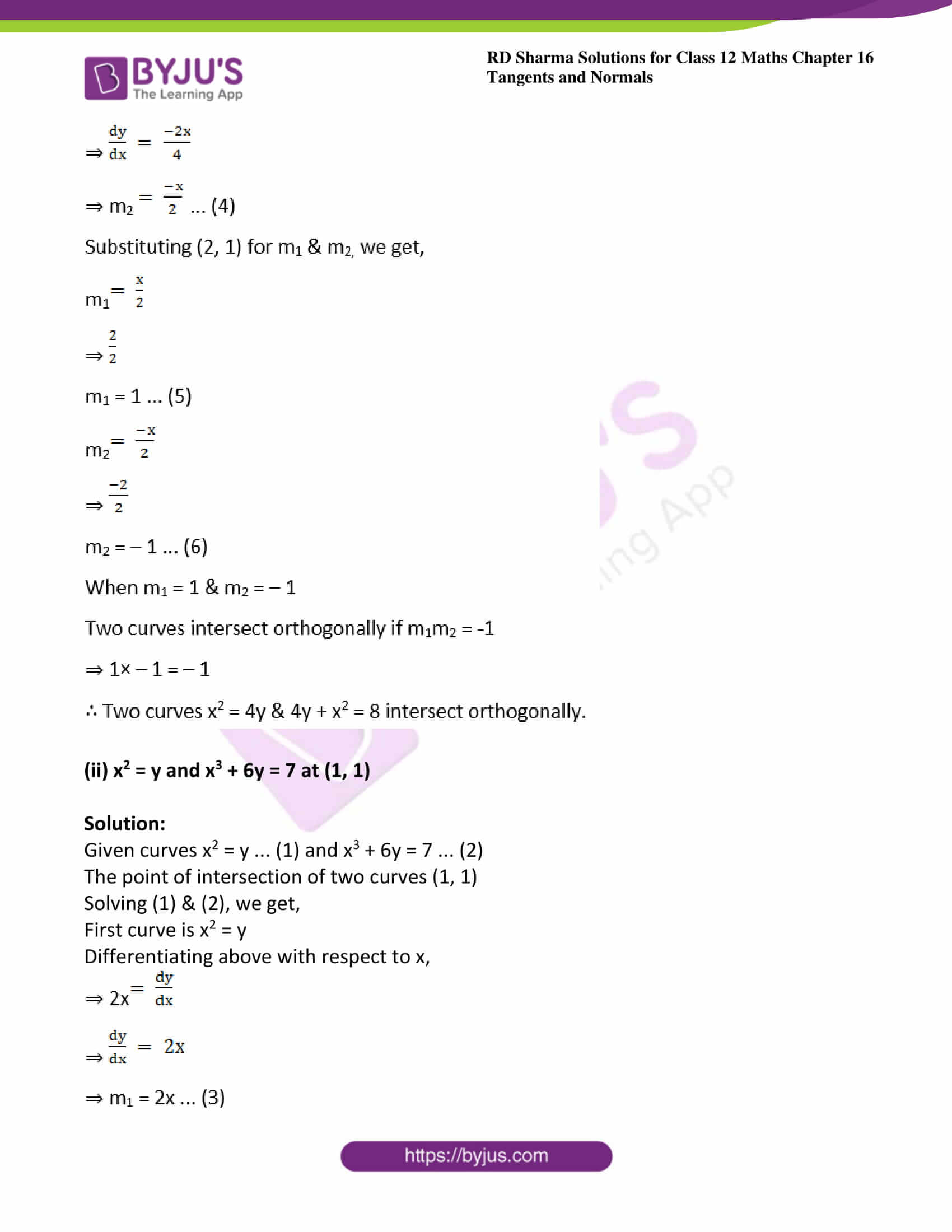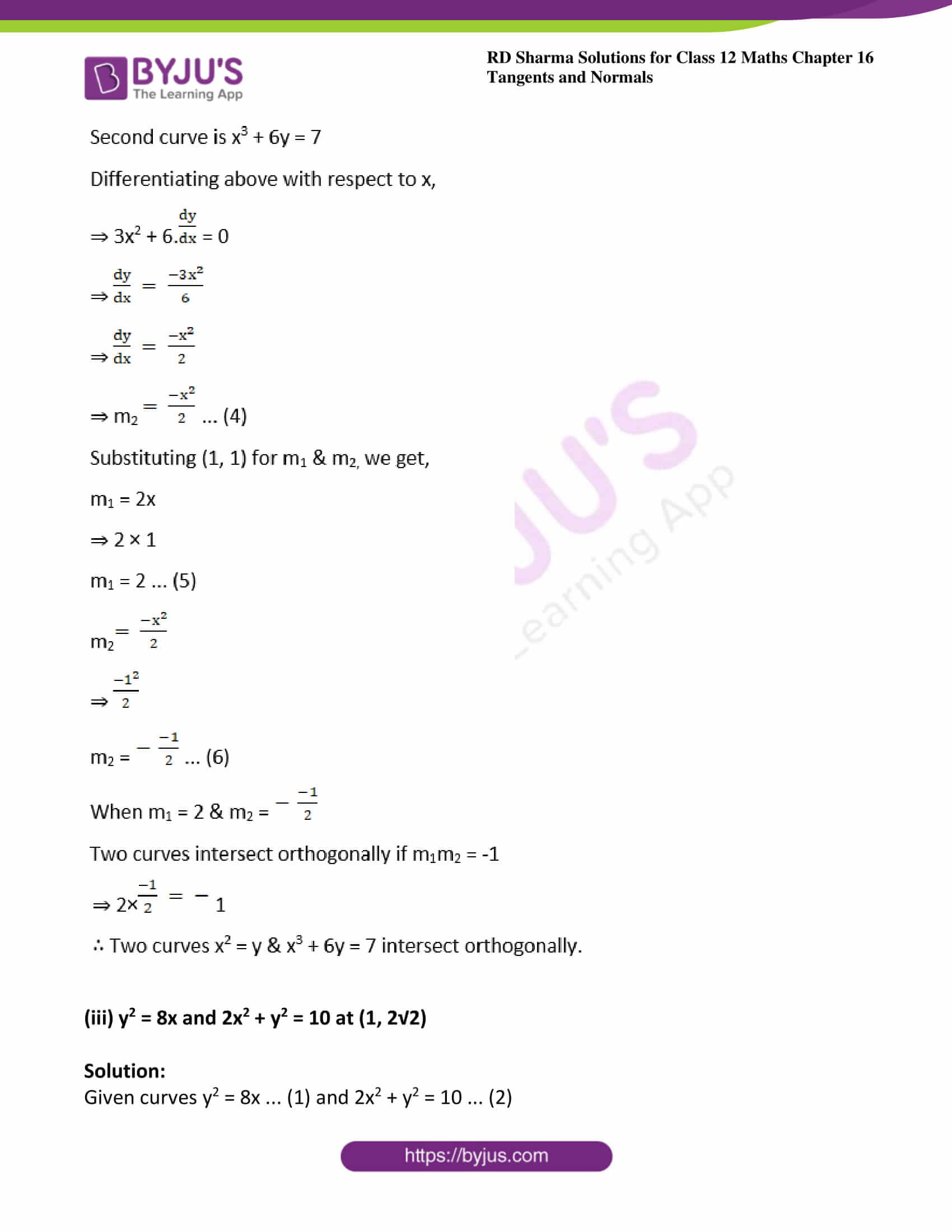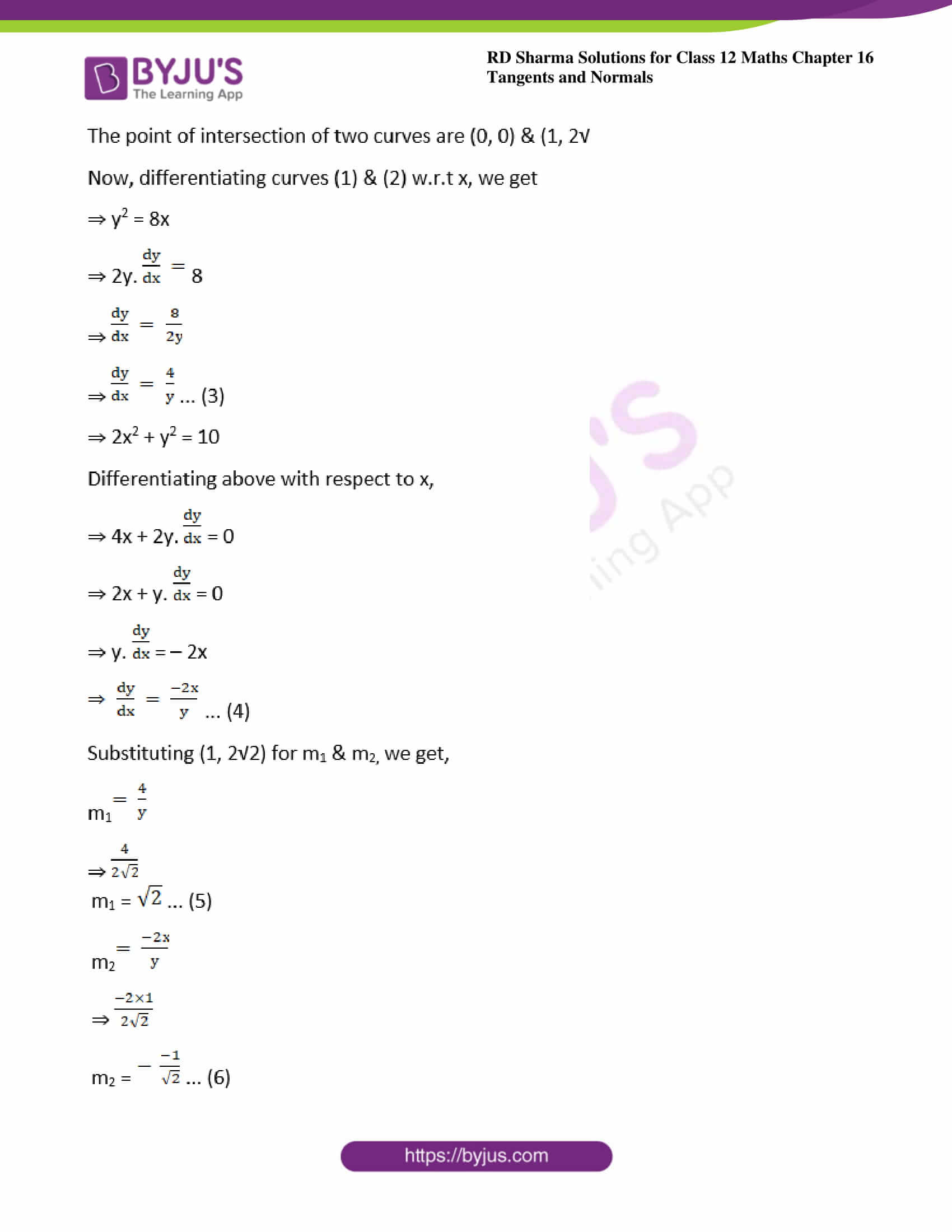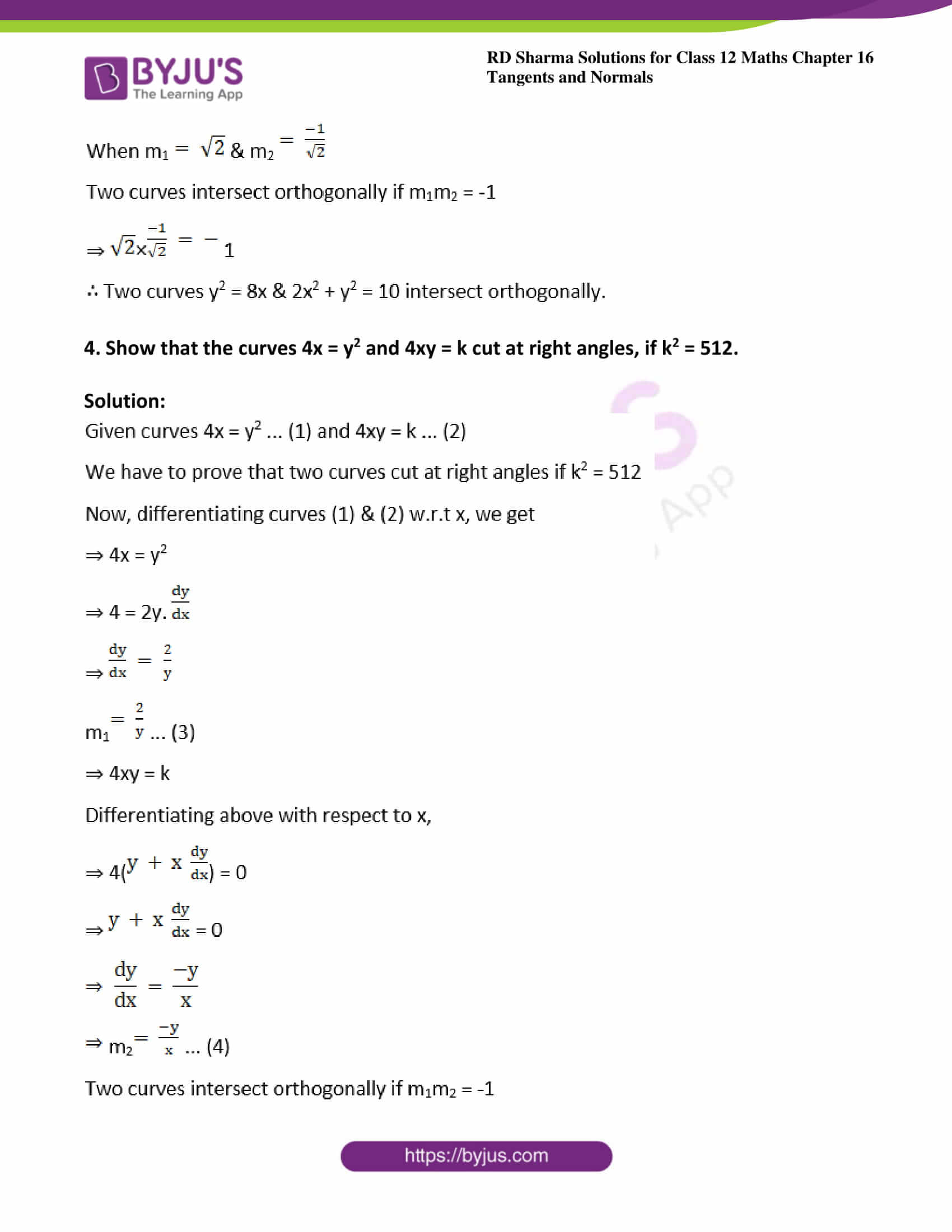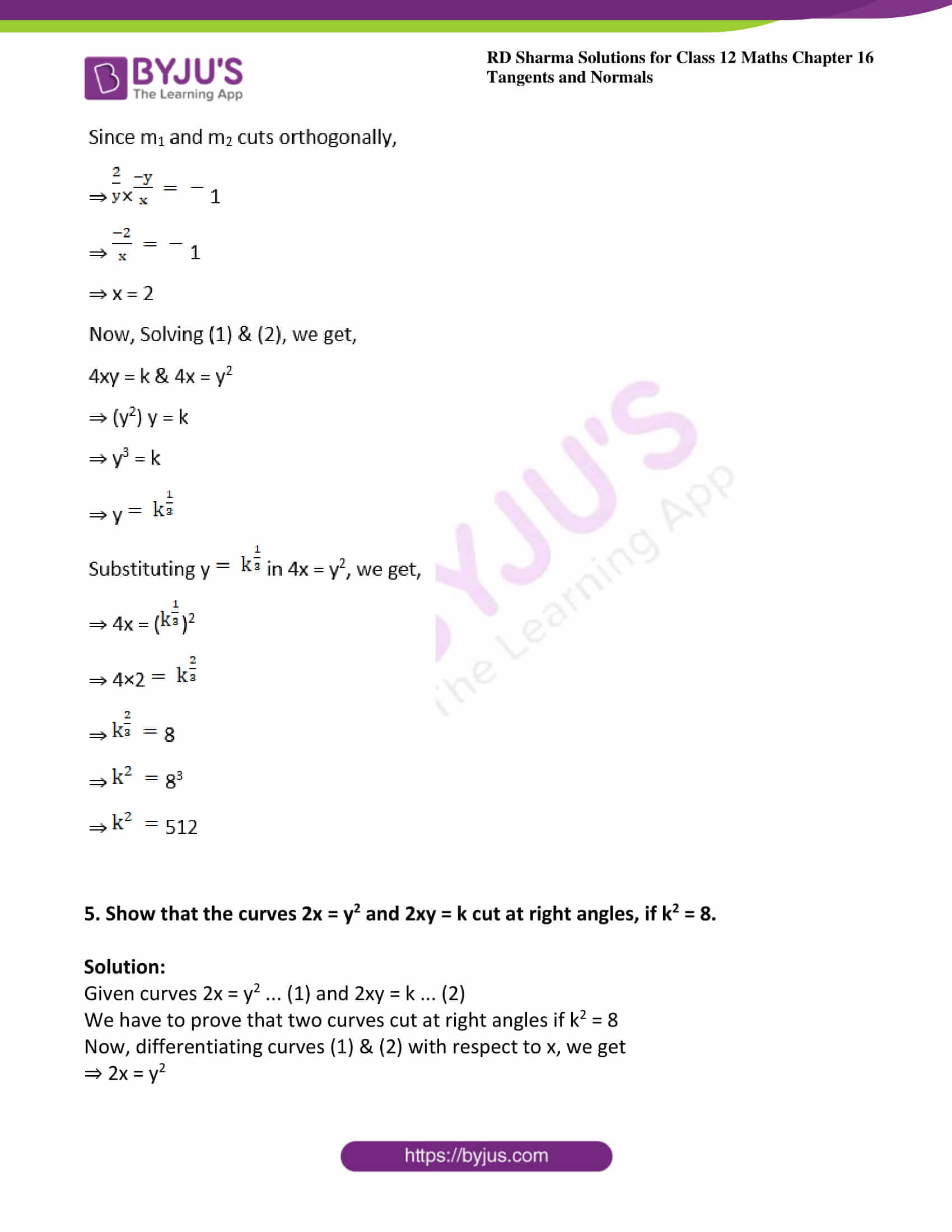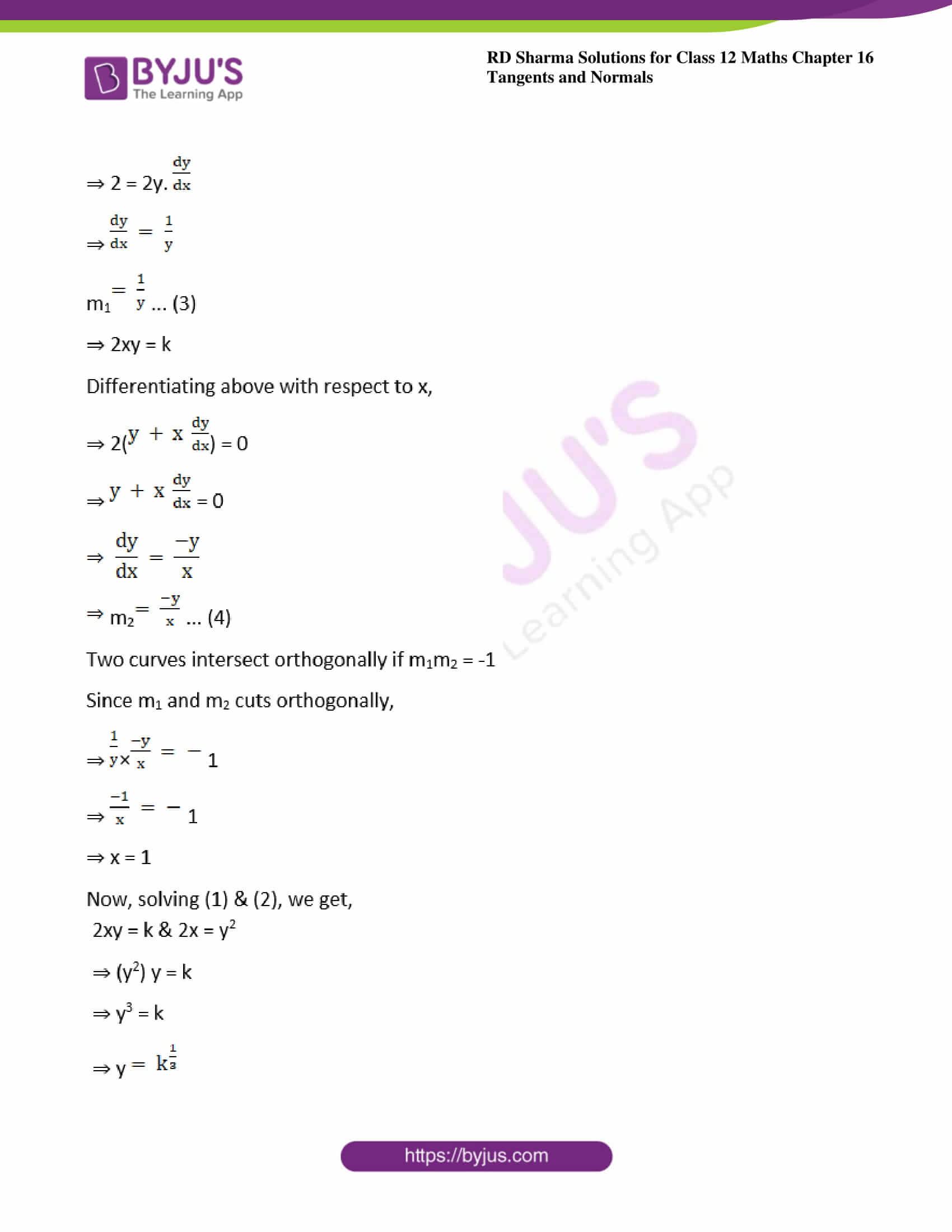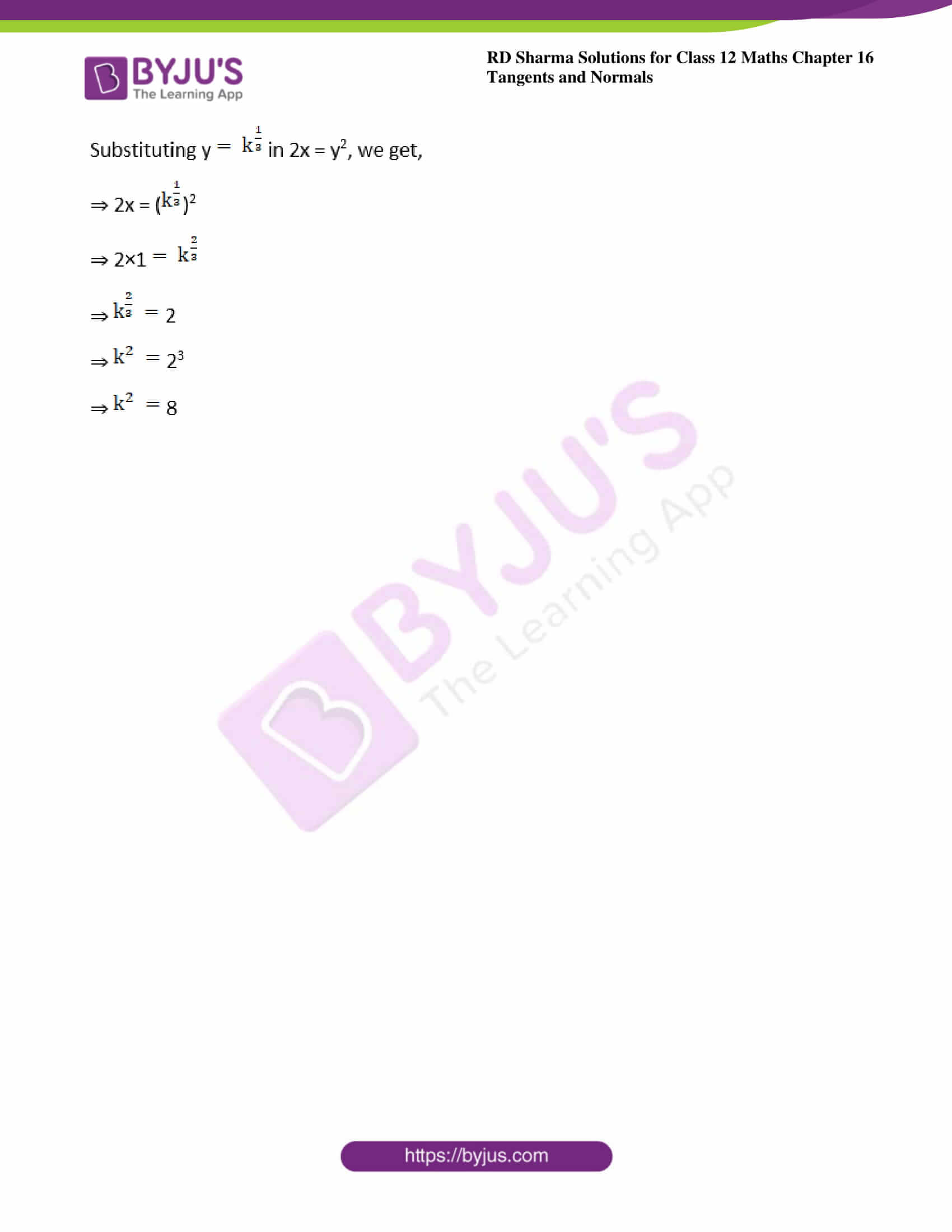### Access other exercises of RD Sharma Solutions For Class 12 Chapter 16 – Tangents and Normals

Exercise 16.1 Solutions

Exercise 16.2 Solutions

### Access answers to Maths RD Sharma Solutions For Class 12 Chapter 16 – Tangents and Normals Exercise 16.3

Exercise 16.3 Page No: 16.40

1. Find the angle to intersection of the following curves:

(i) y2 = x and x2 = y

Solution: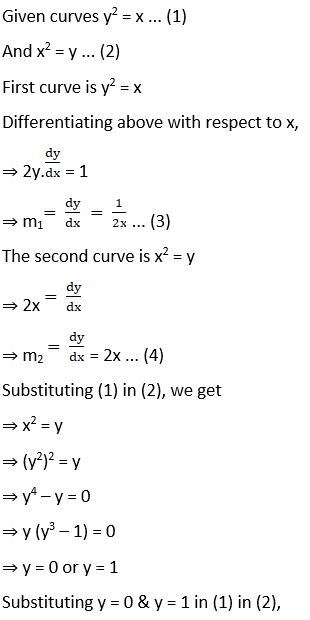x = y2

When y = 0, x = 0

When y = 1, x = 1

Substituting above values for m1 & m2, we get,

When x = 0,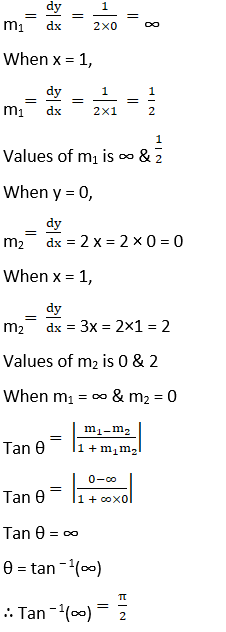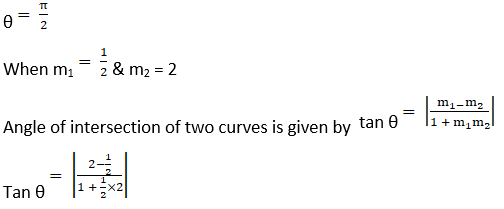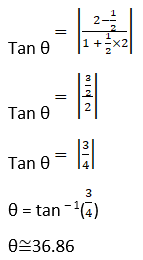(ii) y = x2 and x2 + y2 = 20

Solution: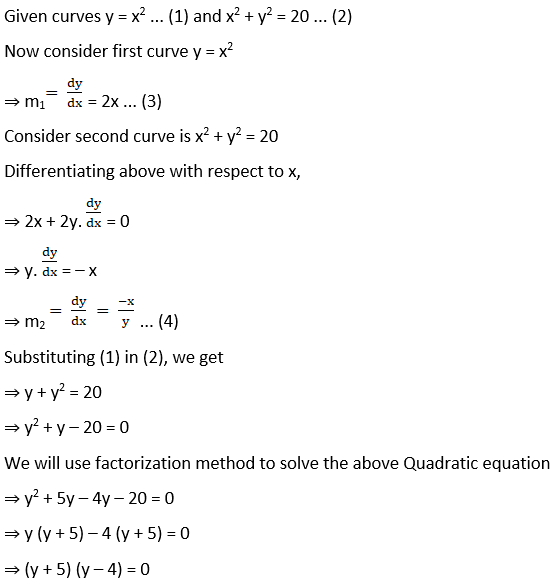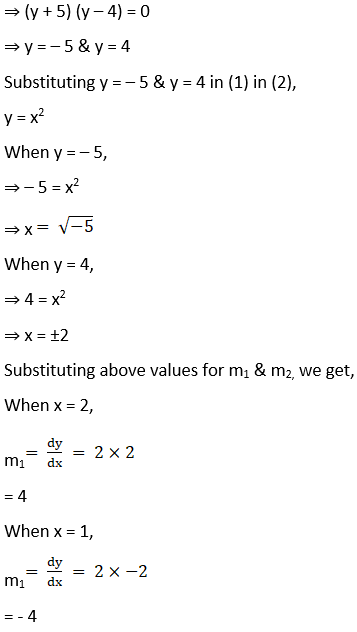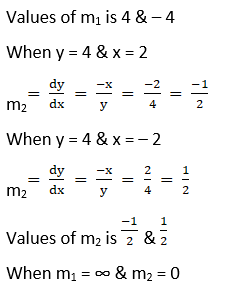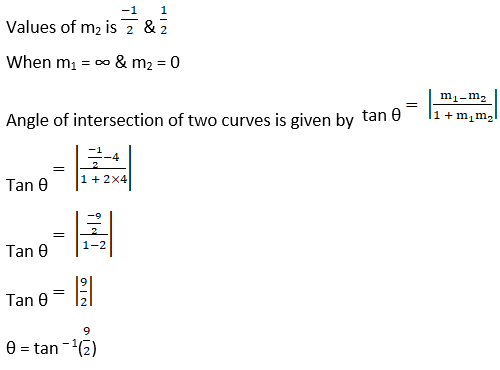(iii) 2y2 = x3 and y2 = 32x

Solution: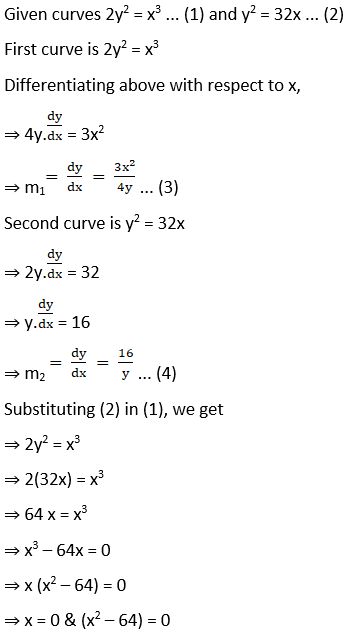Substituting (2) in (1), we get

⇒ 2y2 = x3

⇒ 2(32x) = x3

⇒ 64 x = x3

⇒ x3 – 64x = 0

⇒ x (x2 – 64) = 0

⇒ x = 0 & (x2 – 64) = 0

⇒ x = 0 & ±8

Substituting x = 0 & x = ±8 in (1) in (2),

y2 = 32x

When x = 0, y = 0

When x = 8

⇒ y2 = 32 × 8

⇒ y2 = 256

⇒ y = ±16

Substituting above values for m1 & m2, we get,

When x = 0, y = 16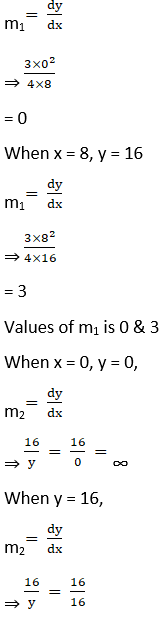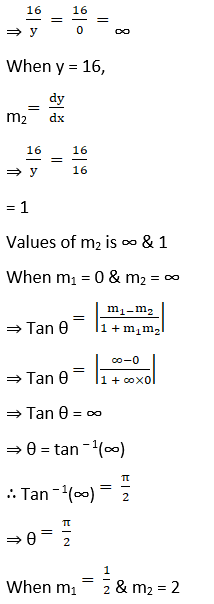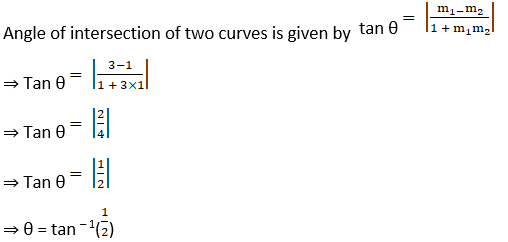(iv) x2 + y2 – 4x – 1 = 0 and x2 + y2 – 2y – 9 = 0

Solution:

Given curves x2 + y2 – 4x – 1 = 0 … (1) and x2 + y2 – 2y – 9 = 0 … (2)

First curve is x2 + y2 – 4x – 1 = 0

⇒ x2 – 4x + 4 + y2 – 4 – 1 = 0

⇒ (x – 2)2 + y2 – 5 = 0

Now, Subtracting (2) from (1), we get

⇒ x2 + y2 – 4x – 1 – ( x2 + y2 – 2y – 9) = 0

⇒ x2 + y2 – 4x – 1 – x2 – y2 + 2y + 9 = 0

⇒ – 4x – 1 + 2y + 9 = 0

⇒ – 4x + 2y + 8 = 0

⇒ 2y = 4x – 8

⇒ y = 2x – 4

Substituting y = 2x – 4 in (3), we get,

⇒ (x – 2)2 + (2x – 4)2 – 5 = 0

⇒ (x – 2)2 + 4(x – 2)2 – 5 = 0

⇒ (x – 2)2(1 + 4) – 5 = 0

⇒ 5(x – 2)2 – 5 = 0

⇒ (x – 2)2 – 1 = 0

⇒ (x – 2)2 = 1

⇒ (x – 2) = ±1

⇒ x = 1 + 2 or x = – 1 + 2

⇒ x = 3 or x = 1

So, when x = 3

y = 2×3 – 4

⇒ y = 6 – 4 = 2

So, when x = 3

y = 2 × 1 – 4

⇒ y = 2 – 4 = – 2

The point of intersection of two curves are (3, 2) & (1, – 2)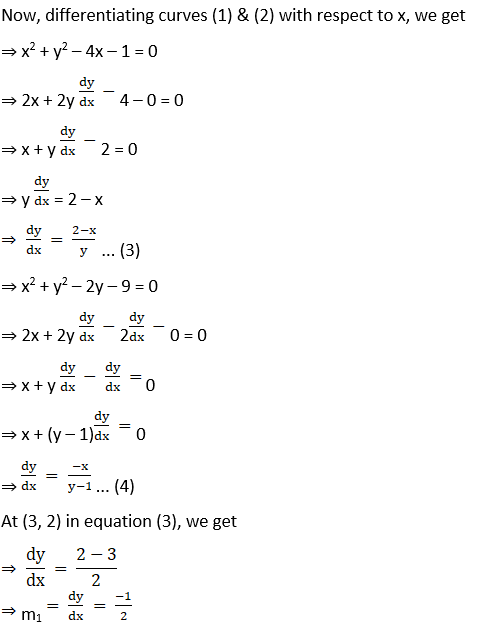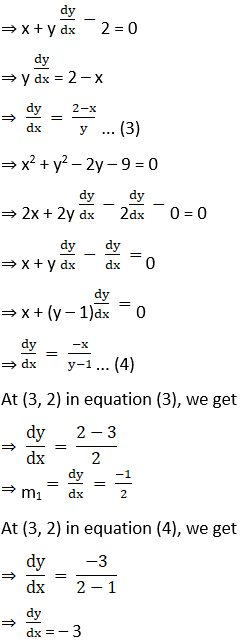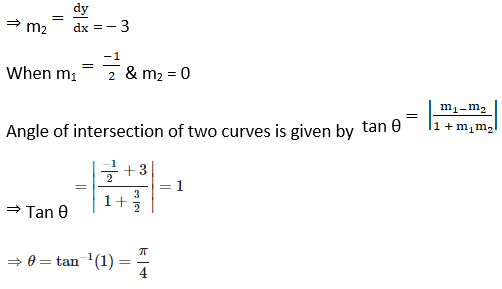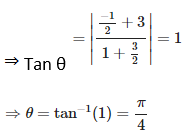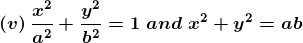Solution: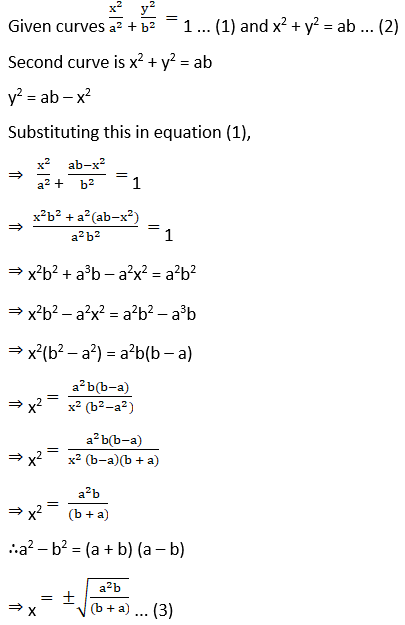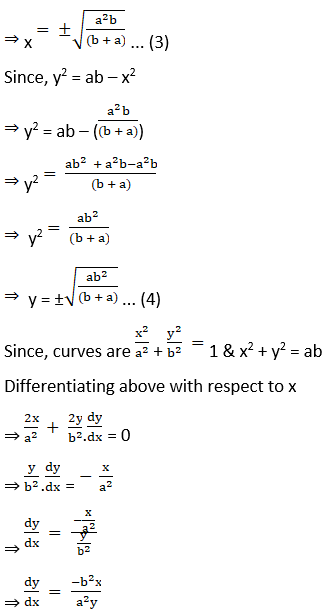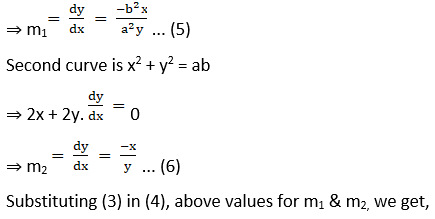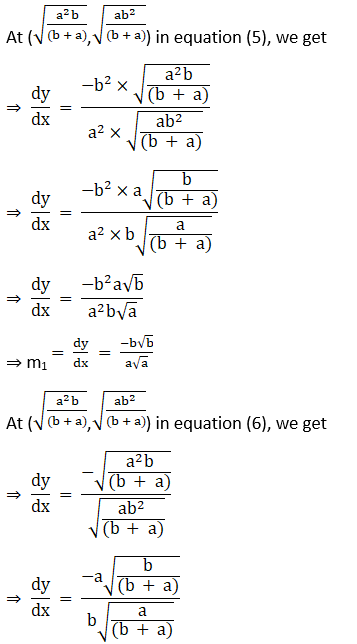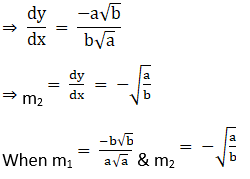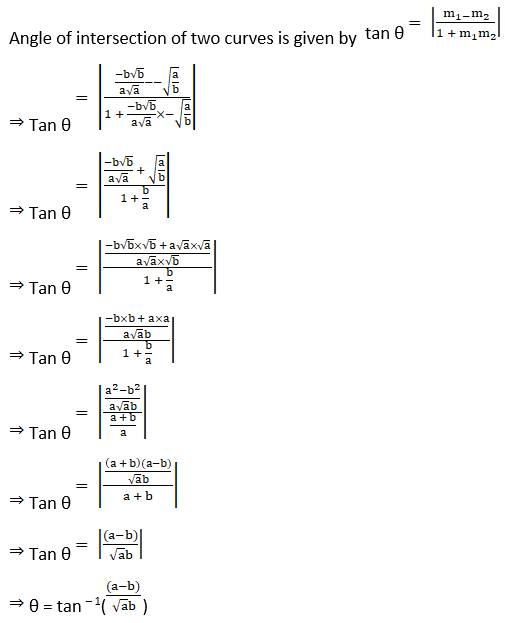2. Show that the following set of curves intersect orthogonally:
(i) y = x3 and 6y = 7 – x2

Solution:

Given curves y = x3 … (1) and 6y = 7 – x2 … (2)

Solving (1) & (2), we get

⇒ 6y = 7 – x2

⇒ 6(x3) = 7 – x2

⇒ 6x3 + x2 – 7 = 0

Since f(x) = 6x3 + x2 – 7,

We have to find f(x) = 0, so that x is a factor of f(x).

When x = 1

f (1) = 6(1)3 + (1)2 – 7

f (1) = 6 + 1 – 7

f (1) = 0

Hence, x = 1 is a factor of f(x).

Substituting x = 1 in y = x3, we get

y = 13

y = 1

The point of intersection of two curves is (1, 1)

First curve y = x3

Differentiating above with respect to x,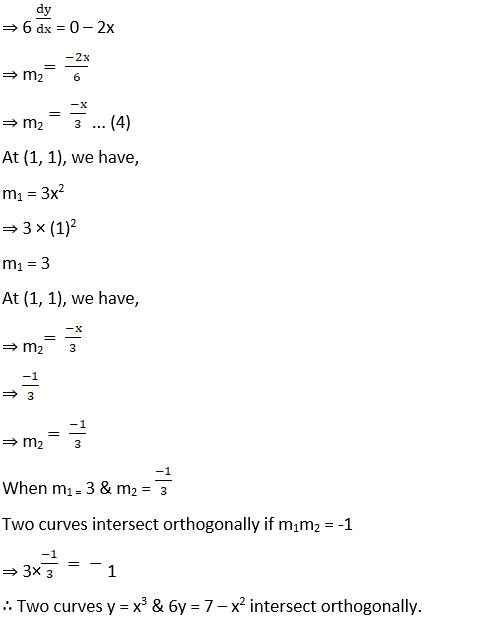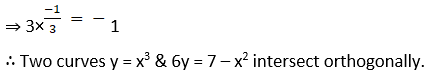(ii) x3 – 3xy2 = – 2 and 3x2 y – y3 = 2

Solution:

Given curves x3 – 3xy2 = – 2 … (1) and 3x2y – y3 = 2 … (2)

Adding (1) & (2), we get

⇒ x3 – 3xy2 + 3x2y – y3 = – 2 + 2

⇒ x3 – 3xy2 + 3x2y – y3 = – 0

⇒ (x – y)3 = 0

⇒ (x – y) = 0

⇒ x = y

Substituting x = y on x3 – 3xy2 = – 2

⇒ x3 – 3 × x × x2 = – 2

⇒ x3 – 3x3 = – 2

⇒ – 2x3 = – 2

⇒ x3 = 1

⇒ x = 1

Since x = y

y = 1

The point of intersection of two curves is (1, 1)

First curve x3 – 3xy2 = – 2

Differentiating above with respect to x,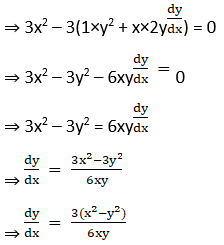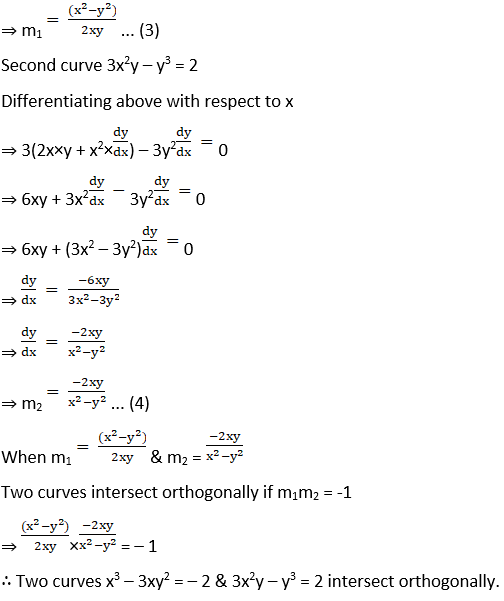(iii) x2 + 4y2 = 8 and x2 – 2y2 = 4.

Solution: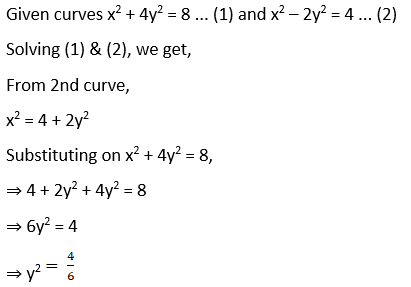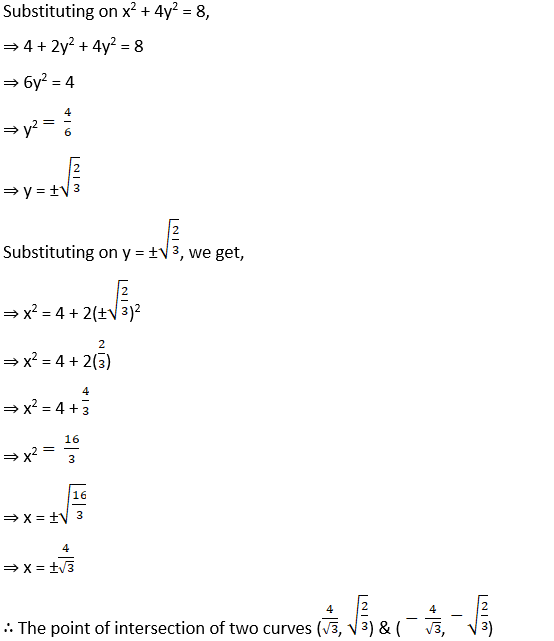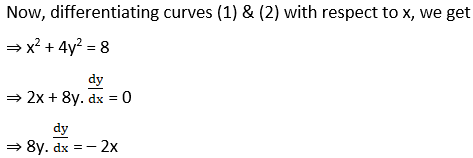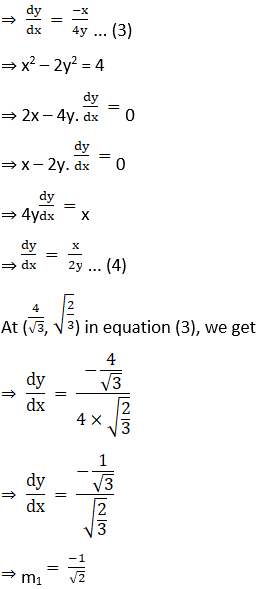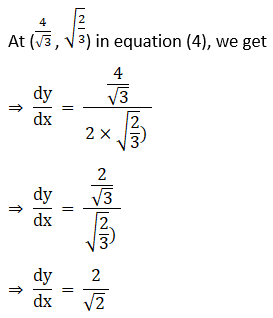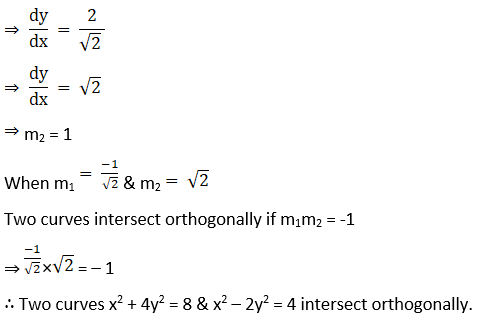3. x2 = 4y and 4y + x2 = 8 at (2, 1)

Solution: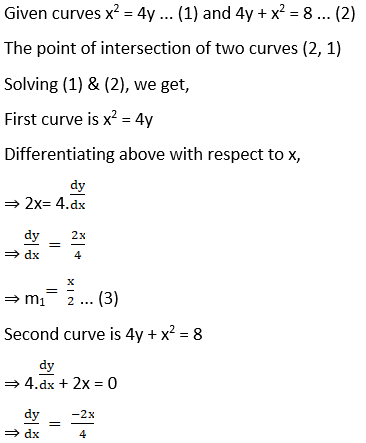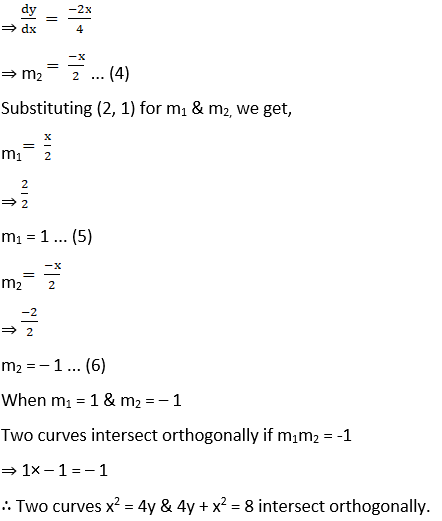(ii) x2 = y and x3 + 6y = 7 at (1, 1)

Solution:

Given curves x2 = y … (1) and x3 + 6y = 7 … (2)

The point of intersection of two curves (1, 1)

Solving (1) & (2), we get,

First curve is x2 = y

Differentiating above with respect to x,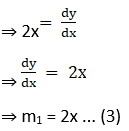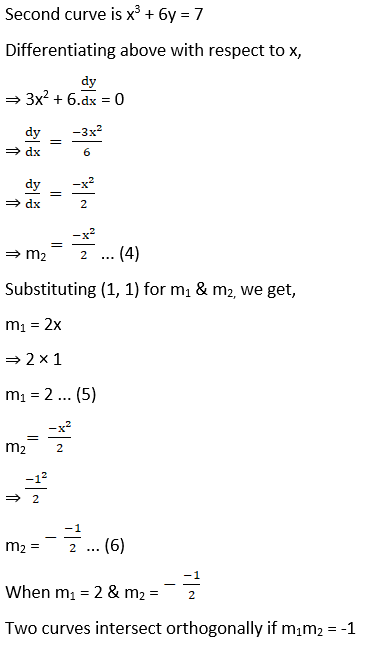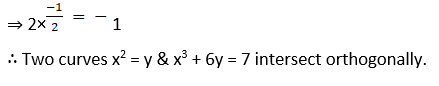(iii) y2 = 8x and 2x2 + y2 = 10 at (1, 2√2)

Solution:

Given curves y2 = 8x … (1) and 2x2 + y2 = 10 … (2)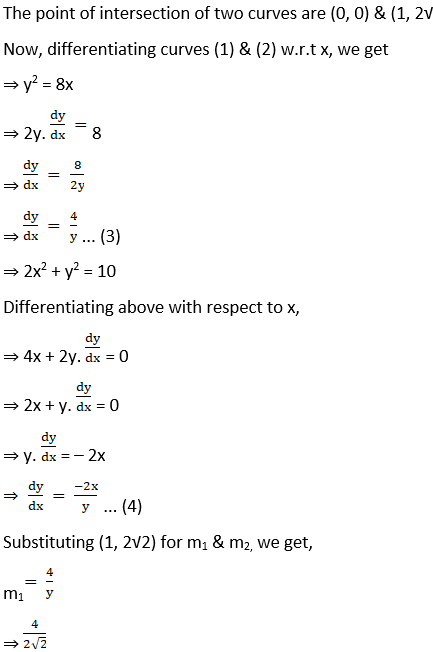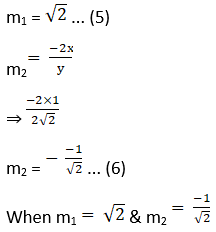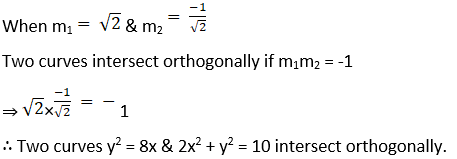4. Show that the curves 4x = y2 and 4xy = k cut at right angles, if k2 = 512.

Solution: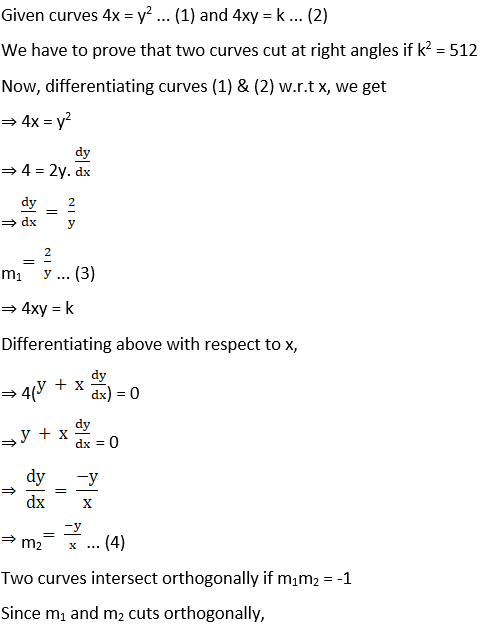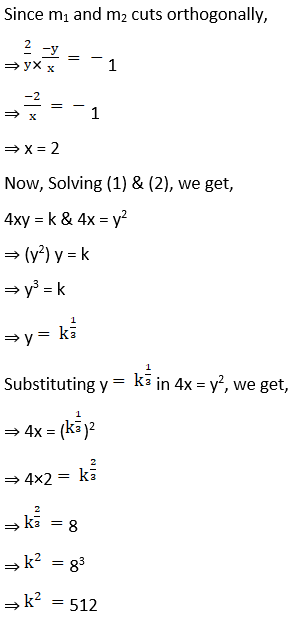5. Show that the curves 2x = y2 and 2xy = k cut at right angles, if k2 = 8.

Solution:

Given curves 2x = y2 … (1) and 2xy = k … (2)

We have to prove that two curves cut at right angles if k2 = 8

Now, differentiating curves (1) & (2) with respect to x, we get

⇒ 2x = y2